Brackets: ( ) page numbers for Dover Edition (Crew and de Salvio) ; [ ] paging in National Edition.

### Two New Sciences, pp. 160-243



NATURALLY ACCELERATED MOTION

The properties belonging to uniform motion have been discussed in the preceding section; but accelerated motion remains to be considered.

And first of all it seems desirable to find and explain a definition best fitting natural phenomena.  For anyone may invent an arbitrary type of motion and discuss its properties; thus, for instance, some have imagined helices and conchoids as described by certain motions which are not met with in nature, and have very commendably established the properties which these curves possess in virtue of their definitions; but we have decided to consider the phenomena of bodies falling with an acceleration such as actually occurs in nature and to make this definition of accelerated motion exhibit the essential features of observed accelerated motions.  And this, at last, after repeated efforts we trust we have succeeded in doing.  In this belief we are confirmed mainly by the consideration that experimental results are seen to agree with and exactly correspond with those properties which have been, one after another, demonstrated by us.  Finally, in the investigation of naturally accelerated motion we were led, by hand as it were, in following the habit and custom of (161) nature herself, in all her various other processes, to employ only those means which are most common, simple and easy.

For I think no one believes that swimming or flying can be accomplished in a manner simpler or easier than that instinctively employed by fishes and birds.

When, therefore, I observe a stone initially at rest falling from an elevated position and continually acquiring new increments of speed, why should I not believe that such increases take place in a manner which is exceedingly simple and rather obvious to everybody?  If now we examine the matter carefully we find no addition or increment more simple than that which repeats itself always in the same manner.  This we readily understand when we consider the intimate relationship between time and motion; for just as uniformity of motion is defined by and conceived through equal times and equal spaces (thus we call a motion uniform when equal distances are traversed during equal time-intervals), so also we may, in a similar manner, through equal time-intervals, conceive additions of speed as taking place without complication; thus we may picture to our  mind a motion as uniformly and continuously accelerated when, during any equal intervals of time whatever, equal increments of speed are given to it.  Thus if any equal intervals of time whatever have elapsed, counting from the time at which the moving body left its position of rest and began to descend, the amount of speed acquired during the first two time-intervals will be double that acquired during the first time-interval alone; so the amount added during three of these time-intervals will be treble; and that in four, quadruple that of the first time interval.  To put the matter more clearly, if a body were to continue its motion with the same speed which it had acquired during the first time-interval and were to retain this same uniform speed, then its motion would be twice as slow as that which it would have if its velocity had been acquired during two time intervals.

And thus, it seems, we shall not be far wrong if we put the increment of speed as proportional to the increment of time; (162) hence the definition of motion which we are about to discuss may be stated as follows: A motion is said to be uniformly accelerated, when starting from rest, it acquires, during equal time-intervals, equal increments of speed.

SAGR.   Although I can offer no rational objection to this or indeed to any other definition, devised by any author whomsoever, since all definitions are arbitrary, I may nevertheless without offense be allowed to doubt whether such a definition as the above, established in an abstract manner, corresponds to and describes that kind of accelerated motion which we meet in nature in the case of freely falling bodies.  And since the Author apparently maintains that the motion described in his definition is that of freely falling bodies, I would like to clear my mind of certain difficulties in order that I may later apply myself more earnestly to the propositions and their demonstrations.

SALV.   It is well that you and Simplicio raise these difficulties.  They are, I imagine, the same which occurred to me when I first saw this treatise, and which were removed either by discussion with the Author himself, or by turning the matter over in my own mind.

SAGR.   When I think of a heavy body falling from rest, that is, starting with zero speed and gaining speed in proportion to the  time from the beginning of the motion; such a motion as would, for instance, in eight beats of the pulse acquire eight degrees of speed; having at the end of the fourth beat acquired four degrees; at the end of the second, two; at the end of the first, one: and since time is divisible without limit, it follows from all these considerations that if the earlier speed of a body is less than its present speed in a constant ratio, then there is no degree of speed however small (or, one may say, no degree of slowness however great) with which we may not find this body travelling after starting from infinite slowness, i.e., from rest.  So that if that speed which it had at the end of the fourth beat was such that, if kept uniform, the body would traverse two miles in an hour, and if keeping the speed which it had at the end of the (163) second beat, it would traverse one mile an hour, we must infer that, as the instant of starting is more and more nearly approached, the body moves so slowly that, if it kept on moving at this rate, it would not traverse a mile in an hour, or in a day, or in a year or in a thousand years; indeed, it would not traverse a span in an even greater time; a phenomenon which baffles the imagination, while our senses show us that a heavy falling body suddenly acquires great speed.

SALV.   This is one of the difficulties which I also at the beginning, experienced, but which I shortly afterwards removed; and the removal was effected by the very experiment which creates the difficulty for you.  You say the experiment appears to show that immediately after a heavy body starts from rest it acquires a very considerable speed: and I say that the same experiment makes clear the fact that the initial motions of a falling body, no matter how heavy, are very slow and gentle.  Place a heavy body upon a yielding material, and leave it there without any pressure except that owing to its own weight; it is clear that if one lifts this body a cubit or two and allows it to fall upon the same material, it will, with this impulse, exert a new and greater pressure than that caused by its mere weight; and this effect is brought about by the [weight of the] falling body together with the velocity acquired during the fall, an effect which will be greater and greater according to the height of the fall, that is according as the velocity of the falling body becomes greater.  From the quality and intensity of the blow we are thus enabled to accurately estimate the speed of a falling body.  But tell me, gentlemen, is it not true that if a block be allowed to fall upon a stake from a height of four cubits and drives it into the earth,  say, four finger-breadths, that coming from a height of two cubits it will drive the stake a much less distance, and from the height of one cubit a still less distance; and finally if the block be lifted only one finger-breadth how much more will it accomplish than if merely laid on top of the stake without percussion?  Certainly very little.  If it be lifted only the thickness of a leaf, the effect will be altogether imperceptible.  And since the (164) effect of the blow depends upon the velocity of this striking body, can any one doubt the motion is very slow and the speed more than small whenever the effect [of the blow] is imperceptible?  See now the power of truth; the same experiment which at first glance seemed to show one thing, when more carefully examined, assures us of the contrary.

But without depending upon the above experiment, which is doubtless very conclusive, it seems to me that it ought not to be difficult to establish such a fact by reasoning alone.  Imagine a heavy stone held in the air at rest; the support is removed and the stone set free; then since it is heavier than the air it begins to fall, and not with uniform motion but slowly at the beginning and with a continuously accelerated motion.  Now since velocity can be increased and diminished without limit, what reason is there to believe that such a moving body starting with infinite slowness, that is, from rest, immediately acquires a speed of ten degrees rather than one of four, or of two, or of one, or of a half, or of a hundredth; or, indeed, of any of the infinite number of small values [of speed]?  Pray listen.  I hardly think you will refuse to grant that the gain of speed of the stone falling from rest follows the same sequence as the diminution and loss of this same speed when, by some impelling force, the stone is thrown to its former elevation: but even if you do not grant this, I do not see how you can doubt that the ascending stone, diminishing in speed, must before coming to rest pass through every possible degree of slowness.

SIMP.   But if the number of degrees of greater and greater slowness is limitless, they will never be all exhausted, therefore such an ascending heavy body will never reach rest, but will continue to move without limit always at a slower rate; but this is not the observed fact.

SALV.   This would happen, Simplicio, if the moving body were to maintain its speed for any length of time at each degree of velocity; but it merely passes each point without delaying more than an instant: and since each time-interval however  small may be divided into an infinite number of instants, these (165) will always be sufficient [in number] to correspond to the infinite degrees of diminished velocity.

That such a heavy rising body does not remain for any length of time at any given degree of velocity is evident from the following: because if, some time-interval having been assigned, the body moves with the same speed in the last as in the first instant of that time-interval, it could from this second degree of elevation be in like manner raised through an equal height, just as it was transferred from the first elevation to the second, and by the same reasoning would pass from the second to the third and would finally continue in uniform motion forever.

SAGR.   From these considerations it appears to me that we may obtain a proper solution of the problem discussed by philosophers, namely, what causes the acceleration in the natural motion of heavy bodies?  Since, as it seems to me, the force [virtù] impressed by the agent projecting the body upwards diminishes continuously, this force, so long as it was greater than the contrary force of gravitation, impelled the body upwards; when the two are in equilibrium the body ceases to rise and passes through the state of rest in which the impressed impetus [impeto] is not destroyed, but only its excess over the weight of the body has been consumed -- the excess which caused the body to rise.  Then as the diminution of the outside impetus [impeto] continues, and gravitation gains the upper hand, the fall begins, but slowly at first on account of the opposing impetus [virtù impressa], a large portion of which still remains in the body; but as this continues to diminish it also continues to be more and more overcome by gravity, hence the continuous acceleration of motion.

SIMP.   The idea is clever, yet more subtle than sound; for even if the argument were conclusive, it would explain only the case in which a natural motion is preceded by a violent motion, in which there still remains active a portion of the external force [virtù esterna]; but where there is no such remaining portion and the body starts from an antecedent state of rest, the cogency of the whole argument fails.

SAGR.   I believe that you are mistaken and that this distinction (166) between cases which you make is superfluous or rather nonexistent.  But, tell me, cannot a projectile receive from the projector either a large or a small force [virtù] such as will throw it to a height of a hundred cubits, and even twenty or four or one?  

SIMP.   Undoubtedly, yes.

SAGR.   So therefore this impressed force [virtù impressa] may exceed the resistance of gravity so slightly as to raise it only a finger-breadth; and finally the force [virtù] of the projector may be just large enough to exactly balance the resistance of gravity so that the body is not lifted at all but merely sustained.  When one holds a stone in his hand does he do anything but give it a force impelling [virtù impellente] it upwards equal to the power [facoltà] of gravity drawing it downwards?  And do you not continuously impress this force [virtù] upon the stone as long as you hold it in the hand?  Does it perhaps diminish with the time during which one holds the stone?

And what does it matter whether this support which prevents the stone from falling is furnished by one's hand or by a table or by a rope from which it hangs?  Certainly nothing at all.  You must conclude, therefore, Simplicio, that it makes no difference whatever whether the fall of the stone is preceded by a period of rest which is long, short, or instantaneous provided only the fall does not take place so long as the stone is acted upon by a force [virtù] opposed to its weight and sufficient to hold it at rest.

SALV.   The present does not seem to be the proper time to investigate the cause of the acceleration of natural motion concerning which various opinions have been expressed by various philosophers, some explaining it by attraction to the center, others to repulsion between the very small parts of the body, while still others attribute it to a certain stress in the surrounding medium which closes in behind the falling body and drives it from one of its positions to another.  Now, all these fantasies, and others too, ought to be examined; but it is not really worth while.  At present it is the purpose of our Author merely to (167) investigate and to demonstrate some of the properties of accelerated motion (whatever the cause of this acceleration may be) -- meaning thereby a motion, such that the momentum of its velocity [i momenti delta sua velocità] goes on increasing after departure from rest, in simple proportionality to the time, which is the same as saying that in equal time-intervals the bodv receives equal increments of velocity; and if we find the properties [of accelerated motion] which will be demonstrated later are realized in freely falling and accelerated bodies, we may conclude that the assumed definition includes such a motion of falling bodies and that their speed [accelerazione] goes on increasing as the time and the duration of the motion.  

SAGR.   So far as I see at present, the definition might have been put a little more clearly perhaps without changing the fundamental idea, namely, uniformly accelerated motion is such that its speed increases in proportion to the space traversed; so that, for example, the speed acquired by a body in falling four cubits would be double that acquired in falling two cubits and this latter speed would be double that acquired in the first cubit.  Because there is no doubt but that a heavy body falling from the height of six cubits has, and strikes with, a momentum [impeto] double that it had at the end of three cubits, triple that which it had at the end of one.

SALV.   It is very comforting to me to have had such a companion in error; and moreover let me tell you that your proposition seems so highly probable that our Author himself admitted, when I advanced this opinion to him, that he had for some time shared the same fallacy.  But what most surprised me was to see two propositions so inherently probable that they commanded the assent of everyone to whom they were presented, proven in a few simple words to be not only false, but impossible.

SIMP.   I am one of those who accept the proposition, and believe that a falling body acquires force [vires] in its descent, its velocity increasing in proportion to the space, and that the momentum [momento] of the falling body is doubled when it falls (168) from a doubled height; these propositions, it appears to me, ought to be conceded without hesitation or controversy.

SALV.   And yet they are as false and impossible, as that motion should be completed instantaneously; and here is a very clear demonstration of it.  If the velocities are in proportion to the spaces traversed, or to be traversed, then these spaces are traversed in equal intervals of time; if, therefore, the velocity with which the falling body traverses a space of eight feet were double that with which it covered the first four feet (just as the one distance is double the other) then the time-intervals required for these passages would be equal.  But for one and the same body to fall eight feet and four feet in the same time is possible only in the case of instantaneous [discontinuous] motion;  but observation shows us that the motion of a falling body occupies time, and less of it in covering a distance of four feet than of eight feet; therefore it is not true that its velocity increases in proportion to the space.

The falsity of the other proposition may be shown with equal clearness.  For if we consider a single striking body the difference of momentum in its blows can depend only upon difference of velocity; for if the striking body falling from a double height were to deliver a blow of double momentum, it would be necessary for this body to strike with a doubled velocity; but with this doubled speed it would traverse a doubled space in the same time-interval; observation however shows that the time required for fall from the greater height is longer.

SAGR.   You present these recondite matters with too much evidence and ease; this great facility makes them less appreciated than they would be had they been presented in a more abstruse manner.  For, in my opinion, people esteem more lightly that knowledge which they acquire with so little labor than that acquired through long and obscure discussion.

SALV.   If those who demonstrate with brevity and clearness the fallacy of many popular beliefs were treated with contempt instead of gratitude the injury would be quite bearable; but on the other hand it is very unpleasant and annoying to see men, (169) who claim to be peers of anyone in a certain field of study, take for granted certain conclusions which later are quickly and easily shown by another to be false.  I do not describe such a feeling as one of envy, which usually degenerates into hatred and anger against those who discover such fallacies; I would call it a strong desire to maintain old errors, rather than accept newly discovered truths.  This desire at times induces them to unite against these truths, although at heart believing in them, merely for the purpose of lowering the esteem in which certain others are held by the unthinking crowd.  Indeed, I have heard from our Academician many such fallacies held as true but easily refutable; some of these I have in mind.

SAGR.   You must not withhold them from us, but, at the proper time, tell us about them even though an extra session be necessary.  But now, continuing the thread of our talk, it would  seem that up to the present we have established the definition of uniformly accelerated motion which is expressed as follows:

A motion is said to be equally or uniformly accelerated when, starting from rest, its momentum (celeritatis momenta) receives equal increments in equal times.

SALV.   This definition established, the Author makes a single assumption, namely,

The speeds acquired by one and the same body moving down planes of different inclinations are equal when the heights of these planes are equal.

By the height of an inclined plane we mean the perpendicular let fall from the upper end of the plane upon the horizontal line drawn through the lower end of the same plane.  Thus, to illustrate, let the line AB be horizontal, and let the planes CA and CD be inclined to it; then the Author calls the perpendicular CB the "height" of the planes CA and CD; he supposes that the speeds acquired by one and the same body, descending along the planes CA and CD to the terminal points A and D are equal since the heights of these planes are the same, CB; and also it must be understood that this speed is that which would be acquired by the same body falling from C to B.  (170)

SAGR.   Your assumption appears to me so reasonable that it ought to be conceded without question, provided of course there are no chance or outside resistances, and that the planes are hard and smooth, and that the figure of the moving body is perfectly round, so that neither plane nor moving body is rough.  All resistance and opposition having been removed, my reason tells me at once that a heavy and perfectly round ball descending along the lines CA, CD, CB would reach the terminal points A, D, B, with equal momenta [impeti eguali].SALV.   Your words are very plausible; but I hope by experiment to increase the probability to an extent which shall be little short of a rigid demonstration.  

Imagine this page to represent a vertical wall, with a nail driven into it; and from the nail let there be suspended a lead bullet of one or two ounces by means of a fine vertical thread, AB, say from four to six feet long, on this wall draw a horizontal line DC, at right angles to the vertical thread AB, which hangs about two finger-breadths in front of the wall.  Now bring the thread AB with the attached ball into the position AC and set it free; first it will be observed to descend along the arc CBD, to pass the point B, and to travel along the arc BD, till it almost reaches the horizontal CD, a slight shortage being caused by the resistance of the air and the string; from this we may rightly infer that the ball in its descent through the arc CB acquired a momentum [impeto] on reaching B, which was just sufficient to carry it through a similar arc BD to the same height.  Having repeated this experiment many times, let us now drive a nail into the wall close to the perpendicular AB, say at E or F, so that it projects out some five or six finger-breadths in order that the thread, again carrying the bullet through the arc CB, may strike upon the nail E when the bullet reaches B, and thus compel it to traverse the arc BG, described about E as center.  From this (171) we can see what can be done by the same momentum [impeto] which previously starting at the same point B carried the same body through the arc BD to the horizontal CD.  Now, gentlemen, you will observe with pleasure that the ball swings to the point G in the horizontal, and you would see the same thing happen if the obstacle were placed at some lower point, say at F, about which the ball would describe the arc BI, the rise of the ball always terminating exactly on the line CD.  But when the nail is placed so low that the remainder of the thread below it will not reach to the height CD (which would happen if the nail were placed nearer B than to the intersection of AB with thehorizontal CD) then the thread leaps over the nail and twists itself about it.

This experiment leaves no room for doubt as to the truth of our supposition; for since the two arcs CB and DB are equal and similarly placed, the momentum [momento] acquired by the fall through the arc CB is the same as that gained by fall through the arc DB; but the momentum [momento] acquired at B, owing to fall through CB, is able to lift the same body [mobile] through the arc BD; therefore, the momentum acquired in the fall BD is equal to that which lifts the same body through the same arc from B to D; so, in general, every momentum acquired by fall (172) through an arc is equal to that which can lift the same body through the same arc.  But all these momenta [momenti] which cause a rise through the arcs BD, BG, and BI are equal, since they are produced by the same momentum, gained by fall through CB, as experiment shows.  Therefore all the momenta gained by fall through the arcs DB, GB, IB are equal.

SAGR.   The argument seems to me so conclusive and the experiment so well adapted to establish the hypothesis that we may, indeed, consider it as demonstrated.

SALV.   I do not wish, Sagredo, that we trouble ourselves too much about this matter, since we are going to apply this principle mainly in motions which occur on plane surfaces, and not upon curved, along which acceleration varies in a manner greatly different from that which we have assumed for planes.

So that, although the above experiment shows us that the descent of the moving body through the arc CB confers upon it momentum [momento] just sufficient to carry it to the same height through any of the arcs BD, BG, BI, we are not able, by similar means, to show that the event would be identical in the case of a perfectly round ball descending along planes whose inclinations are respectively the same as the chords of these arcs.  It seems likely, on the other hand, that, since these planes form angles at the point B, they will present an obstacle to the ball which has descended along the chord CB, and starts to rise along the chord BD, BG, BI.

In striking these planes some of its momentum [impeto] will be lost and it will not be able to rise to the height of the line CD; but this obstacle, which interferes with the experiment, once removed, it is clear that the momentum [impeto] (which gains  in strength with descent) will be able to carry the body to the same height.  Let us then, for the present, take this as a postulate, the absolute truth of which will be established when we find that the inferences from it correspond to and agree perfectly with experiment.  The author having assumed this single principle passes next to the propositions which he clearly demonstrates; the first of these is as follows: (173)

THEOREM I, PROPOSITION I

The time in which any space is traversed by a body starting from rest and uniformly accelerated is equal to the time in which that same space would be traversed by the same body moving at a uniform speed whose value is the mean of the highest speed and the speed just before acceleration began.

Let us represent by the line AB the time in which the space CD is traversed by a body which starts from rest at C and is uniformly accelerated; let the final and highest value of the speed gained during the interval AB be represented by the line EB, drawn at right angles to AB; draw the line AE, then all lines drawn from equidistant points on AB and parallel to BE will represent the increasing values of the speed, beginning with the instant A.  Let the point F bisect the line EB; draw FG parallel to BA, and GA parallel to FB, thus forming a parallelogram AGFB which will be equal in area to the triangle AEB, since the side GF bisects the side AE at the point I; for if the parallel lines in the triangle AEB are extended to GI, then the sum of all the parallels contained in the quadrilateral is equal to the sum of those contained in the triangle AEB; for those in the triangle IEF are equal to those contained in the triangle GIA, while those included in the trapezium AIFB are common.  Since each and every instant of time in the time-interval AB has its corresponding point on the line AB, from which points parallels drawn in and limited by the triangle AEB represent the increasing values of the growing velocity, and since parallels contained within the rectangle represent the values of a speed which is not increasing, but constant, it appears, in like manner, that the momenta [momenta] assumed by the moving body may also be represented, in the case of the accelerated motion, by the increasing parallels of the triangle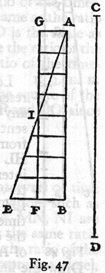(174)  AEB, and, in the case of the uniform motion, by the parallels of the rectangle GB.  For, what the momenta may lack in the first part of the accelerated motion (the deficiency of the momenta being represented by the parallels of the triangle AGI) is made up by the momenta represented by the parallels of the triangle IEF.

Hence it is clear that equal spaces will be traversed in equal times by two bodies, one of which, starting from rest, moves with a uniform acceleration, while the momentum of the other, moving with uniform speed, is one-half its maximum momentum under accelerated motion.  Q.E.D.THEOREM II, PROPOSITION II

The spaces described by a body falling from rest with a uniformly accelerated motion are to each other as the squares of the time-intervals employed in traversing these distances.

Let the time beginning with any instant A be represented by the straight line AB in which are taken any two time-intervals AD and A. E.  Let HI represent the distance through which the body, starting from rest at H, falls with uniform acceleration.  If HL represents the space traversed during the time-interval AD, and HM that covered during the interval AE, then the space MH stands to the space LH in a ratio which is the square of the ratio of the time AE to the time AD; or we may say simply that the distances HM and HL are related as the squares of AE and AD.

Draw the line AC making any angle whatever with the line AB; and from the points D and E, draw the parallel lines DO and EP; of these two lines, DO represents the greatest velocity attained during the interval AD, while EP represents the maximum velocity acquired during the interval AE.  But it has just been proved that so far as distances traversed are concerned (175) it is precisely the same whether a body falls from rest with a uniform acceleration or whether it falls during an equal time-interval with a constant speed which is one-half the maximum speed attained during the accelerated motion.  It follows therefore that the distances HM and HL are the same as would be traversed, during the time-intervals AE and AD, by uniform velocities equal to one-half those represented by DO and EP respectively.  If, therefore, one can show that the distances HM and HL are in the same ratio as the squares of the time-intervals AE and AD, our proposition will be proven.  

But in the fourth proposition of the first book [p.  157 above] it has been shown that the spaces traversed by two particles in uniform motion bear to one another a ratio which is equal to the product of the ratio of the velocities by the ratio of the times.  But in this case the ratio of the velocities is the same as the ratio of the time-intervals (for the ratio of AE to AD is the same as that of ½ EP to ½ DO or of EP to DO).  Hence the ratio of the spaces traversed is the same as the squared ratio of the time intervals.  Q.E.D.

Evidently then the ratio of the distances is the square of the ratio of the final velocities, that is, of the lines EP and DO, since these are to each other as AE to AD.

COROLLARY I

Hence it is clear that if we take any equal intervals of time whatever, counting from the beginning of the motion, such as AD, DE, EF, FG, in which the spaces HL, LM, MN, NI are traversed, these spaces will bear to one another the same ratio as the series of odd numbers, 1, 3, 5, 7; for this is the ratio of the differences of the squares of the lines [which represent time], differences which exceed one another by equal amounts, this excess being equal to the smallest line [viz.  the one representing a single time-interval]: or we may say [that this is the ratio] of the differences of the squares of the natural numbers beginning with unity.  (176)

While, therefore, during equal intervals of time the velocities increase as the natural numbers, the increments in the distances traversed during these equal time-intervals are to one another as the odd numbers beginning with unity.

SAGR.   Please suspend the discussion for a moment since there just occurs to me an idea which I want to illustrate by means of a diagram in order that it may be clearer both to you and to me.

Let the line AI represent the lapse of time measured from the initial instant A; through A draw the straight line AF making any angle whatever; join the terminal points I and F; divide the time AI in half at C; draw CB parallel to IF.  Let us consider CB as the maximum value of the velocity which increases from zero at the beginning, in simple proportionality to the intercepts on the triangle ABC of lines drawn parallel to BC; or what is the same thing, let us suppose the velocity to increase in proportion to the time; then I admit without question, in view of the preceding argument, that the space described by a body falling in the aforesaid manner will be equal to the space traversed by the same body during the same length of time travelling with a uniform speed equal to EC, the half of BC.  Further let us imagine that thebody has fallen with accelerated motion so that, at the instant C, it has the velocity BC.  It is clear that if the body continued to descend with the same speed BC, without acceleration, it would in the next time-interval CI traverse double the distance covered during the interval AC, with the uniform speed EC which is half of BC; but since the falling body acquires equal increments of speed during equal increments of time, it follows that the velocity BC, during the next time- (177) interval CI will be increased by an amount represented by the parallels of the triangle BFG which is equal to the triangle ABC.  If, then, one adds to the velocity GI half of the velocity FG, the highest speed acquired by the accelerated motion and determined by the parallels of the triangle BFG, he will have the uniform velocity with which the same space would have been described in the time CI; and since this speed IN is three times as great as EC it follows that the space described during the interval CI is three times as great as that described during the interval AC.  Let us imagine the motion extended over another equal time-interval IO, and the triangle extended to APO; it is then evident that if the motion continues during the interval IO, at the constant rate IF acquired by acceleration during the time AI, the space traversed during the interval IO will be four times that traversed during the first interval AC, because the speed IF is four times the speed EC.  But if we enlarge our triangle so as to include FPQ which is equal to ABC, still assuming the acceleration to be constant, we shall add to the uniform speed an increment RQ, equal to EC; then the value of the equivalent uniform speed during the time-interval IO will be five times that during the first time-interval AC; therefore the space traversed will be quintuple that during the first interval AC.  It is thus evident by simple computation that a moving body starting from rest and acquiring velocity at a rate proportional to the time, will, during equal intervals of time, traverse distances which are related to each other as the odd numbers beginning with unity, 1, 3, 5;* or considering the total space traversed, that covered  in double time will be quadruple that covered during unit time; in triple time, the space is nine times as great as in unit time.  (178) And in general the spaces traversed are in the duplicate ratio of the times, i.e., in the ratio of the squares of the times.

SIMP.   In truth, I find more pleasure in this simple and clear argument of Sagredo than in the Author's demonstration which to me appears rather obscure; so that I am convinced that matters are as described, once having accepted the definition of uniformly accelerated motion.  But as to whether this acceleration is that which one meets in nature in the case of falling bodies, I am still doubtful; and it seems to me, not only for my own sake but also for all those who think as I do, that this would be the proper moment to introduce one of those experiments -- and there are many of them, I understand -- which illustrate in several ways the conclusions reached.

SALV.   The request which you, as a man of science, make, is a very reasonable one; for this is the custom -- and properly so --- in those sciences where mathematical demonstrations are applied to natural phenomena, as is seen in the case of perspective, astronomy, mechanics, music, and others where the principles, once established by well-chosen experiments, become the foundations of the entire superstructure.  I hope therefore it will not appear to be a waste of time if we discuss at considerable length this first and most fundamental question upon which hinge numerous consequences of which we have in this book only a small number, placed there by the Author, who has done so much to open a pathway hitherto closed to minds of speculative turn.  So far as experiments go they have not been neglected by the Author; and often, in his company, I have attempted in the following manner to assure myself that the acceleration actually experienced by falling bodies is that above described.

A piece of wooden moulding or scantling, about 12 cubits long, half a cubit wide, and three finger-breadths thick, was taken; on its edge was cut a channel a little more than one finger in breadth; having made this groove very straight, smooth, and polished, and having lined it with parchment, also as smooth and polished as possible, we rolled along it a hard, smooth, and very round bronze ball.  Having placed this  (179) board in a sloping position, by lifting one end some one or two cubits above the other, we rolled the ball, as I was just saying, along the channel, noting, in a manner presently to be described, the time required to make the descent.  We repeated this experiment more than once in order to measure the time with an accuracy such that the deviation between two observations never exceeded one-tenth of a pulse-beat.  Having performed this operation and having assured ourselves of its reliability, we now rolled the ball only one-quarter the length of the channel; and having measured the time of its descent, we found it precisely one-half of the former.  Next we tried other distances, comparing the time for the whole length with that for the half, or with that for two-thirds, or three-fourths, or indeed for any fraction; in such experiments, repeated a full hundred times, we always found that the spaces traversed were to each other as the squares of the times, and this was true for all inclinations of the plane, i.e., of the channel, along which we rolled the ball.  We also observed that the times of descent, for various inclinations of the plane, bore to one another precisely that ratio which, as we shall see later, the Author had predicted and demonstrated for them.

For the measurement of time, we employed a large vessel of water placed in an elevated position; to the bottom of this vessel was soldered a pipe of small diameter giving a thin jet of water, which we collected in a small glass during the time of each descent, whether for the whole length of the channel or for a part of its length; the water thus collected was weighed, after each descent, on a very accurate balance; the differences and ratios of these weights gave us the differences and ratios of the times, and this with such accuracy that although the operation was repeated many, many times, there was no appreciable discrepancy in the results.

SIMP.   I would like to have been present at these experiments; but feeling confidence in the care with which you performed them, and in the fidelity with which you relate them, I am satisfied and accept them as true and valid.

SALV.   Then we can proceed without discussion.   (180)

COROLLARY II

Secondly, it follows that, starting from any initial point, if we take any two distances, traversed in any time-intervals whatsoever, these time-intervals bear to one another the same ratio as one of the distances to the mean proportional of the two distances.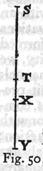For if we take two distances ST and SY measured from T the initial point S, the mean proportional of which is SX, the time of fall through ST is to the time of fall through SY as ST is to SX; or one may say the time of fall through SY is to the time of fall through ST as SY is to SX.  Now since it has been shown that the spaces traversed are in the same ratio as the squares of the times; and since, moreover, the ratio of the space SY to the space ST is the square of the ratio SY to SX, it follows that the ratio of the times of fall through SY and ST is the ratio of the respective distances SY and SX.

SCHOLIUM

The above corollary has been proven for the case of vertical fall; but it holds also for planes inclined at any angle; for it is to be assumed that along these planes the velocity increases in the same ratio, that is, in proportion to the time, or, if you prefer, as the series of natural numbers. *

SALV.   Here, Sagredo, I should like, if it be not too tedious to Simplicio, to interrupt for a moment the present discussion in order to make some additions on the basis of what has already been proved and of what mechanical principles we have already learned from our Academician.  This addition I make for the better establishment on logical and experimental grounds, of the principle which we have above considered; and what is more important, for the purpose of deriving it geometrically, after first demonstrating a single lemma which is fundamental in the science of motion [impeti].  (181)

SAGR.   If the advance which you propose to make is such as will confirm and fully establish these sciences of motion, I will gladly devote to it any length of time.  Indeed, I shall not only  be glad to have you proceed, but I beg of you at once to satisfy the curiosity which you have awakened in me concerning your proposition; and I think that Simplicio is of the same mind.

SIMP.   Quite right.

SALV.   Since then I have your permission, let us first of all consider this notable fact, that the momenta or speeds [i momenti o le velocità] of one and the same moving body vary with the inclination of the plane.

The speed reaches a maximum along a vertical direction, and for other directions diminishes as the plane diverges from the vertical.  Therefore the impetus, ability, energy, [l'impeto, il talento, l'energia] or, one might say, the momentum [il momento] of descent of the moving body is diminished by the plane upon which it is supported and along which it rolls.

For the sake of greater clearness erect the line AB perpendicular to the horizontal AC; next draw AD, AE, AF, etc. , at different inclinations to the horizontal.  Then I say that all the momentum of the falling body is along the vertical and is a maximum when it falls in that direction; the momentum is less along DA and still less along EA, and even less yet along the more inclined plane FA.  Finally on the horizontal plane the momentum vanishes altogether; the body finds itself in a condition of indifference as to motion or rest; has no inherent tendency to move in any direction, and offers no resistance to being set in motion.  For just as a heavy body or system of bodies cannot of itself move upwards, or recede from the common center [comun centro] toward which all heavy things tend, so it is impossible for any body of its own accord to assume any motion other than one which carries it nearer to the aforesaid common center.  Hence, along the horizontal, by which we understand a surface, every point of which is equidistant from this same common center, the body will have no momentum whatever.(182) 

This change of momentum being clear, it is here necessary for me to explain something which our Academician wrote when in Padua, embodying it in a treatise on mechanics prepared solely for the use of his students, and proving it at length and conclusively when considering the origin and nature of that marvelous machine, the screw.  What he proved is the manner in which the momentum [impeto] varies with the inclination of the plane, as for instance that of the plane FA, one end of which is elevated through a vertical distance FC.  This direction FC is that along which the momentum of a heavy body becomes a maximum; let us discover what ratio this momentum bears to that of the same body moving along the inclined plane FA.  This ratio, I say, is the inverse of that of the aforesaid lengths.  Such is the lemma preceding the theorem which I hope to demonstrate a little later.

It is clear that the impelling force [impeto] acting on a body in descent is equal to the resistance or least force [resistenza o forza minima] sufficient to hold it at rest.  In order to measure this force and resistance [forza e resistenza] I propose to use the weight of another body.  Let us place upon the plane FA a body G connected to the weight H by means of a cord passing over the point F; then the body H will ascend or descend, along the perpendicular, the same distance which the body G ascends or descends along the inclined plane FA; but this distance will not be equal to the rise or fall of G along the vertical in which direction alone G, as other bodies, exerts its force [resistenza].  This is clear.  For if we consider the motion of the body G, from A to F, in the triangle AFC to be made up of a horizontal component AC and a vertical component CF, and remember that this body experiences no resistance to motion along the horizontal (because by such  a motion the body neither gains nor loses distance from the common center of heavy things) it follows that resistance is met only in consequence of the body rising through the vertical distance CF.  Since then the body G in moving from A to F offers resistance only in so far as it rises through the vertical distance CF, while the other body H must fall vertically through the entire distance FA, and since this ratio is maintained whether the motion be large or small, the two bodies being inextensibly connected, we are able to assert positively that, in case of equilibrium (bodies at rest) the (183) momenta, the velocities, or their tendency to motion [propensioni al moto], i.e., the spaces which would be traversed by them in equal times, must be in the inverse ratio to their weights.  This is what has been demonstrated in every case of mechanical motion. * So that, in order to hold the weight G at rest, one must give H a weight smaller in the same ratio as the distance CF is smaller than FA.  If we do this, FA:FC = weightG:weightH; then equilibrium will occur, that is, the weights H and G will have the same impelling forces [momenti eguali], and the two bodies will come to rest.

And since we are agreed that the impetus, energy, momentum or tendency to motion of a moving body is as great as the force or least resistance [forza o resistenza minima] sufficient to stop it, and since we have found that the weight H is capable of preventing motion in the weight G, it follows that the less weight H whose entire force [momento totale] is along the perpendicular, FC, will be an exact measure of the component of force [momento parziale] which the larger weight G exerts along the plane FA.  But the measure of the total force [total momento] on the body G is its own weight, since to prevent its fall it is only necessary to balance it with an equal weight, provided this second weight be free to move vertically; therefore the component of the force [momento parziale] on G along the inclined plane FA will bear to the maximum and total force on this same body G along the perpendicular FC the same ratio as the weight H, to the weight G.  This ratio is, by construction, the same which the height, FC, of the inclined plane bears to the length FA.  We have here the lemma which I proposed to demonstrate and which, as you will see, has been assumed by our Author in the second part of the sixth proposition of the present treatise.

SAGR.   From what you have shown thus far, it appears to me that one might infer, arguing ex aequali con la proportione perturbata, that the tendencies [momenti] of one and the same body to move along planes differently inclined, but having the same vertical height, as FA and FI, are to each other inversely as the lengths of the planes.  

SALV.   Perfectly right.  This point established, I pass to the demonstration of the following theorem:

(184) If a body falls freely along smooth planes inclined at any angle whatsoever, but of the same height, the speeds with which it reaches the bottom are the same.

First we must recall the fact that on a plane of any inclination whatever a body starting from rest gains speed or momentum [la quantitá dell'impeto] in direct proportion to the time, in agreement with the definition of naturally accelerated motion given by the Author.  Hence, as he has shown in the preceding proposition, the distances traversed are proportional to the squares of the times and therefore to the squares of the speeds.  The speed relations are here the same as in the motion first studied [i.e., vertical motion], since in each case the gain of speed is proportional to the time.

Let AB be an inclined plane whose height above the level BC is AC.  As we have seen above the force impelling [l'impeto] a body to fall along the vertical AC is to the force which drives the same body along the inclined plane AB as AB is to AC.  On the incline AB, lay off AD a third proportional to AB and AC; then the force producing motion along AC is to that along AB (i.e., along AD) as the length AC is to the length AD.  And therefore the body will traverse the space AD, along the incline AB, in the same time which it would occupy in falling the vertical distance AC, (since the forces [momenti] are in the same ratio as these distances); also the speed at C is to the speed at D as the distance AC is to the distance AD.  But, according to the definition of accelerated motion, the speed at B is to the speed of the same body at D as the time required to traverse AB is to the time required for AD; and, according to the last corollary of the second proposition, the time of passing through the distance AB bears to the time of passing through AD the same ratio as the distance AC (a mean proportional between AB and AD) to AD.  Accordingly the two speeds at B and C each bear to the speed at D the same ratio, namely, that of the distances AC and AD; hence they are equal.  This is the theorem which I set out to prove.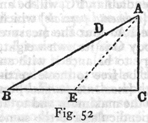From the above we are better able to demonstrate the following third proposition of the Author in which he employs the following principle, namely, the time required to traverse an inclined plane (185) is to that required to fall through the vertical height of the plane in the same ratio as the length of the plane to its height.



For, according to the second corollary of the second proposition, if BA represents the time required to pass over the distance BA, the time required to pass the distance AD will be a mean proportional between these two distances and will be represented by the line AC; but if AC represents the time needed to traverse AD it will also represent the time required to fall through the distance AC, since the distances AC and AD are traversed in equal times; consequently if AB represents the time required for AB then AC will represent the time required for AC.  Hence the times required to traverse AB and AC are to each other as the distances AB and AC.

In like manner it can be shown that the time required to fall through AC is to the time required for any other incline AE as the length AC is to the length AE; therefore, ex aequali, the time of fall along the incline AB is to that along AE as the distance AB is to the distance AE, etc. *

One might by application of this same theorem, as Sagredo will readily see, immediately demonstrate the sixth proposition of the Author; but let us here end this digression which Sagredo has perhaps found rather tedious, though I consider it quite important for the theory of motion.

SAGR.   On the contrary it has given me great satisfaction, and indeed I find it necessary for a complete grasp of this principle.

SALV.   I will now resume the reading of the text.  

THEOREM III, PROPOSITION III

If one and the same body, starting from rest, falls along an inclined plane and also along a vertical, each having the same height, the times of descent will be to each other as the lengths of the inclined plane and the vertical.

Let AC be the inclined plane and AB the perpendicular, each having the same vertical height above the horizontal, namely, BA; then I say, the time of descent of one and the same body (186)  along the plane AC bears a ratio to the time of fall along the perpendicular AB, which is the same as the ratio of the length AC to the length AB.  Let DG, EI and LF be any lines parallel to the horizontal CB; then it follows from what has preceded that a body starting from A will acquire the same speed at the point G as at D, since in each case the vertical fall is the same; in like manner the speeds at I and E will be the same; so also those at L and F.  And in general the speeds at the two extremities of any parallel drawn from any point on AB to the corresponding point on AC will be equal.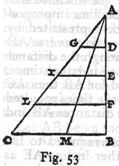Thus the two distances AC and AB are traversed at the same speed.  But it has already been proved  that if two distances are traversed by a body moving with equal speeds, then the ratio of the times of descent will be the ratio of the distances themselves; therefore, the time of descent along AC is to that along AB as the length of the plane AC is to the vertical distance AB.  Q.E.D.



SAGR.   It seems to me that the above could have been proved clearly and briefly on the basis of a proposition already demonstrated, namely, that the distance traversed in the case of accelerated motion along AC or AB is the same as that covered  by a uniform speed whose value is one-half the maximum speed, CB; the two distances AC and AB having been traversed at the same uniform speed it is evident, from Proposition I, that the times of descent will be to each other as the distances.

COROLLARY

Hence we may infer that the times of descent along planes having different inclinations, but the same vertical height stand (187) to one another in the same ratio as the lengths of the planes.  For consider any plane AM extending from A to the horizontal CB; then it may be demonstrated in the same manner that the time of descent along AM is to the time along AB as the distance AM is to AB; but since the time along AB is to that along AC as the length AB is to the length AC, it follows, ex aequali, that as AM is to AC so is the time along AM to the time along AC.

THEOREM IV, PROPOSITION IV

The times of descent along planes of the same length but of different inclinations are to each other in the inverse ratio of the square roots of their heights

From a single point B draw the planes BA and BC, having the same length but different inclinations; let AE and CD be horizontal lines drawn to meet the perpendicular BD; and  let BE represent the height of the plane AB, and BD the height of BC; also let BI be a mean proportional to BD and BE; then the ratio of BD to BI is equal to the square root of the ratio of BD to BE.  Now, I say, the ratio of the times of descent along BA and BC is the ratio of BD to BI; so that the time of descent along BA is related to the height of the other plane BC, namely BD as the time along BC is related to the height BI.  Now it must be proved that the time of descent along BA is to that along BC as the length BD is to the length BI.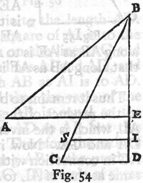Draw IS parallel to DC; and since it has been shown that the time of fall along BA is to that along the vertical BE as BA is to BE; and also that the time along BE is to that along BD as BE is to BI; and likewise that the time along BD is to that along BC as BD is to BC, or as BI to BS; it follows, ex aequali, that the time along BA is to that along BC as BA to BS, or BC to BS.  However, BC is to BS as BD is to BI; hence follows our proposition .

(188)

THEOREM V, PROPOSITION V

The times of descent along planes of different length, slope and height bear to one another a ratio which is equal to the product of the ratio of the lengths by the square root of the inverse ratio of their heights.

Draw the planes AB and AC, having different inclinations, lengths, and heights.  My theorem then is that the ratio of the time of descent along AC to that along AB is equal to the product of the ratio of AC to AB by the square root of the inverse ratio of their heights.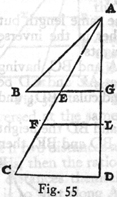For let AD be a perpendicular to which are drawn the horizontal lines BG and CD; also let AL be a mean proportional to the heights AG and AD; from the point L draw a horizontal line meeting AC in F; accordingly AF will be a mean proportional between AC and AE.  Now since the time of descent along AC is to that along AE as the length AF is to AE; and since the time along AE is to that along AB as AE is to AB, it is clear that the time along AC is to that along AB as AF is to AB.



Thus it remains to be shown that the ratio of AF to AB is equal to the product of the ratio of AC to AB by the ratio of AG to AL, which is the inverse ratio of the square roots of the heights DA and GA.  Now it is evident that, if we consider the line AC in connection with AF and AB, the ratio of AF to AC is the same as that of AL to AD, or AG to AL which is the square root of the ratio of the heights AG and AD; but the ratio of AC to AB is the ratio of the lengths themselves.  Hence follows the theorem.

THEOREM VI, PROPOSITION VI

If from the highest or lowest point in a vertical circle there be drawn any inclined planes meeting the circumference the (189) times of descent along these chords are each equal to the other.

On the horizontal line GH construct a vertical circle.  From its lowest point -- the point of tangency with the horizontal -- draw the diameter FA and from the highest point, A, draw inclined planes to B and C, any points whatever on the circumference; then the times of descent along these are equal.  Draw BD and CE perpendicular to the diameter; make AI a mean proportional between the heights of the planes, AE and AD; and since the rectangles FA. AE and FA. AD are respectively equal to the squares of AC and AB, while the rectangle FA. AE is to the rectangle FA. AD as AE is to AD, it follows that the square of AC is to the square of AB as the length AE is to the length AD.  But since the length AE is to AD as the square of AI is to the square of AD, it follows that the squares on the lines AC and AB are to each other as the squares on the lines AI and AD, and hence also the length AC is to the length AB as AI is to AD.  But it has previously been demonstrated that the ratio of the time of descent along AC to that along AB is equal to the product of the two ratios AC to AB and AD to AI; but this last ratio is the same as that of AB to AC.  Therefore the ratio of the time of descent along AC to that along AB is the product of the two ratios, AC to AB and AB to AC.  The ratio of these times is therefore unity.  Hence follows our proposition.By use of the principles of mechanics [ex mechanicis] one may obtain the same result, namely, that a falling body will require equal times to traverse the distances CA and DA, indicated in the following figure.  Lay off BA equal to DA, and let fall the  perpendiculars BE and DF; it follows from the principles of (190) mechanics that the component of the momentum [momentum ponderis] acting along the inclined plane ABC is to the total momentum [i.e., the momentum of the body falling freely] as BE is to BA; in like manner the momentum along the plane AD is to its total momentum [i.e., the momentum of the body falling freely] as DF is to DA, or to BA.  Therefore the momentum of this same weight along the plane DA is to that along the plane ABC as the length DF is to the length BE; for this reason, this same weight will in equal times according to the second proposition of the first book, traverse spaces along the planes CA and DA which are to each other as the lengths BE and DF.  But it can be shown that CA is to DA as BE is to DF.  Hence the falling body will traverse the two paths CA and DA in equal times.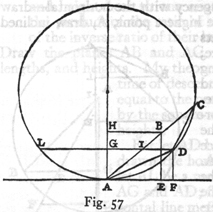Moreover the fact that CA is to DA as BE is to DF may be demonstrated as follows: Join C and D; through D, draw the line DGL parallel to AF and cutting the line AC in I; through B draw the line BH, also parallel to AF.  Then the angle ADI will be equal to the angle DCA, since they subtend equal arcs LA and DA, and since the angle DAC is common, the sides of the triangles, CAD and DAI, about the common angle will be proportional to each other; accordingly as CA is to DA so is DA to IA, that is as BA is to IA, or as HA is to GA, that is as BE is to DF.  E. D.

The same proposition may be more easily demonstrated as follows: On the horizontal line AB draw a circle whose diameter DC is vertical.  From the upper end of this diameter draw any inclined plane, DF, extending to meet the circumference; then, I say, a body will occupy the same time in falling along the plane DF as along the diameter DC.  For draw FG parallel (191) to AB and perpendicular to DC; join FC; and since the time of fall along DC is to that along DG as the mean proportional  between CD and GD is to GD itself; and since also DF is a mean proportional between DC and DG, the angle DFC inscribed in a semicircle being a right-angle, and FG being perpendicular to DC, it follows that the time of fall along DC is to that along DG as the length FD is to GD.  But it has already been demonstrated that the time of descent along DF is to that along DG as the length DF is to DG; hence the times of descent along DF and DC each bear to the time of fall along DG the same ratio; consequently they are equal.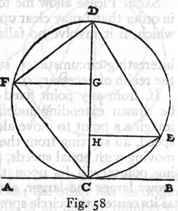In like manner it may be shown that if one draws the chord CE from the lower end of the diameter, also the line EH parallel to the horizon, and joins the points E and D, the time of descent along EC, will be the same as that along the diameter, DC.

COROLLARY I

From this it follows that the times of descent along all chords drawn through either C or D are equal one to another.

COROLLARY II

It also follows that, if from any one point there be drawn a vertical line and an inclined one along which the time of descent is the same, the inclined line will be a chord of a semicircle of which the vertical line is the diameter.

COROLLARY III

Moreover the times of descent along inclined planes will be equal when the vertical heights of equal lengths of these planes (192) are to each other as the lengths of the planes themselves; thus it is clear that the times of descent along CA and DA, in the figure just before the last, are equal, provided the vertical height of AB (AB being equal to AD), namely, BE, is to the vertical height DF as CA is to DA.

SAGR.   Please allow me to interrupt the lecture for a moment in order that I may clear up an idea which just occurs to me; one which, if it involve no fallacy, suggests at least a freakish and  interesting circumstance, such as often occurs in nature and in the realm of necessary consequences.

If, from any point fixed in a horizontal plane, straight lines be drawn extending indefinitely in all directions, and if we imagine a point to move along each of these lines with constant speed, all starting from the fixed point at the same instant and moving with equal speeds, then it is clear that all of these moving points will lie upon the circumference of a circle which grows larger and larger, always having the aforesaid fixed point as its center; this circle spreads out in precisely the same manner as the little waves do in the case of a pebble allowed to drop into quiet water, where the impact of the stone starts the motion in all directions, while the point of impact remains the center of these ever-expanding circular waves.  But imagine a vertical plane from the highest point of which are drawn lines inclined at every angle and extending indefinitely; imagine also that heavy particles descend along these lines each with a naturally accelerated motion and each with a speed appropriate to the inclination of its line.  If these moving particles are always visible, what will be the locus of their positions at any instant?  Now the answer to this question surprises me, for I am led by the preceding theorems to believe that these particles will always lie upon the circumference of a single circle, ever increasing in size as the particles recede farther and farther from the point at which their motion began.  To be more definite, let A be the fixed point from which are drawn the lines AF and AH inclined at any angle whatsoever.  On the perpendicular AB take any two points C and D about which, as centers, circles are described (193) passing through the point A, and cutting the inclined lines at the points F, H, B, E, G, I.  From the preceding theorems it is clear that, if particles start, at the same instant, from A and descend along these lines, when one is at E another will be at G and another at I; at a later instant they will be found simultaneously at F, H and B; these, and indeed an infinite number of other particles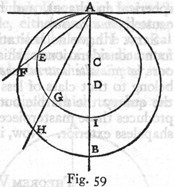travelling along an infinite number of different slopes will at successive instants always lie upon a single ever-expanding circle.  The two kinds of motion occurring in nature give rise therefore to two infinite series of circles, at once resembling and differing from each other; the one takes its rise in the center of an infinite number of concentric circles; the other has its origin in the contact, at their highest points, of an infinite number of eccentric circles; the former are produced by motions which are equal and uniform; the latter by motions which are neither uniform nor equal among themselves, but which vary from one to another according to the slope.

Further, if from the two points chosen as origins of motion, we draw lines not only along horizontal and vertical planes but in all directions then just as in the former cases, beginning at a single point ever-expanding circles are produced, so in the latter case an infinite number of spheres are produced about a single point, or rather a single sphere which expands in size without limit; and this in two ways, one with the origin at the center, the other on the surface of the spheres.

SALV.   The idea is really beautiful and worthy of the clever mind of Sagredo.

SIMP.   As for me, I understand in a general way how the two kinds of natural motions give rise to the circles and spheres; and yet as to the production of circles by accelerated motion and its proof, I am not entirely clear; but the fact that one can take (194) the origin of motion either at the inmost center or at the very top of the sphere leads one to think that there may be some great mystery hidden in these true and wonderful results, a mystery related to the creation of the universe (which is said to be spherical in shape), and related also to the seat of the first cause [prima causa].

SALV.   I have no hesitation in agreeing with you.  But profound considerations of this kind belong to a higher science than ours [a più alte dottrine che le nostre].  We must be satisfied to belong to that class of less worthy workmen who procure from the quarry the marble out of which, later, the gifted sculptor produces those masterpieces which lay hidden in this rough and shapeless exterior.  Now, if you please, let us proceed.  

THEOREM VII, PROPOSITION VII

If the heights of two inclined planes are to each other in the same ratio as the squares of their lengths, bodies starting from rest will traverse these planes in equal times.

Take two planes of different lengths and different inclinations, AE and AB, whose heights are AF and AD: let AF be to AD as the square of AE is to the square of AB; then, I say, that a body, starting from rest at A, will traverse the planes AE and AB in equal times.  From the vertical line, draw the horizontal parallel lines EF and DB, the latter cutting AE at G.  Since FA:DA = EA2:BA2, and since FA:DA = EA:GA, it follows that EA:GA = EA2:BA2.  Hence BA is a mean proportional between EA and GA.  Now since the time of descent along AB bears to the time along AG the same ratio which AB bears to AG and since also the time of descent along AG is to the time along AE as AG is to a mean proportional between AG and AE, that is, to AB, it follows, ex aequali,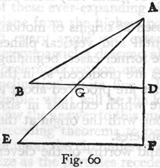(195) that the time along AB is to the time along AE as AB is to itself.  Therefore the times are equal.  Q.E.D.

THEOREM VIII, PROPOSITION VIII

The times of descent along all inclined planes which intersect one and the same vertical circle, either at its highest or lowest point, are equal to the time of fall along the vertical diameter; for those planes which fall short of this diameter the times are shorter; for planes which cut this diameter, the times are longer.

Let AB be the vertical diameter of a circle which touches the horizontal plane.  It has already been proven that the times of descent along planes drawn from either end, A or B, to the circumference are equal.  In order to show that the time of descent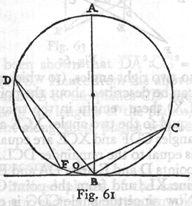along the plane DF which falls short of the diameter is shorter we may draw the plane DB which is both longer and less steeply inclined than DF; whence it follows that the time along DF is less than that along DB and consequently along AB.  In like manner, it is shown that the time of descent along CO which cuts the diameter is greater: for it is both longer and less steeply inclined than CB.  Hence follows the theorem.

THEOREM IX, PROPOSITION IX

If from any point on a horizontal line two planes, inclined at any angle, are drawn, and if they are cut by a line which makes with them angles alternately equal to the angles between these planes and the horizontal, then the times required to traverse those portions of the plane cut off by the aforesaid line are equal.  (196)

Through the point C on the horizontal line X, draw two planes CD and CE inclined at any angle whatever: at any point in the line CD lay off the angle CDF equal to the angle XCE; let the line DF cut CE at F so that the angles CDF and CFD are alternately equal to XCE and LCD; then, I say, the times of descent over CD and CF are equal.  Now since the angle CDF is equal to the angle XCE by construction, it is evident that the angle CFD must be equal to the angle DCL.  For if the common angle DCF be subtracted from the three angles of the triangle CDF, together equal to two right angles, (to which are also equal all the angles which can be described about the point C on the lower side of the line LX) there remain in the triangle two angles, CDF and CFD, equal to the two angles XCE and LCD; but, by hypothesis, the angles CDF and XCE are equal; hence the remaining angle CFD is equal to the remainder DCL.  Take CE equal to CD; from the points D and E draw DA and EB perpendicular to the horizontal line XL; and from the point C draw CG perpendicular to DF.  Now since the angle CDG is equal to the angle ECB and since DGC and CBE are right angles, it follows that the triangles CDG and CBE are equiangular; consequently DC:CG=CE:EB.  But DC is equal to CE, and therefore CG is equal to EB.  Since also the angles at C and at A, in the triangle DAC, are equal to the angles at F and G in the triangle CGF, we have CD:DA= FC:CG and, permutando, DC:CF=DA:CG=DA:BE.  Thus the ratio of the heights of the equal planes CD and CE is the same as the ratio of the lengths DC and CF.  Therefore, by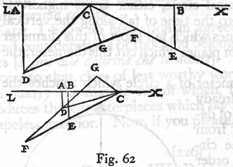Corollary I of Prop.  VI, the times of descent along these planes will be equal.  Q.E.D.

An alternative proof is the following: Draw FS perpendicular (197) to the horizontal line AS.  Then, since the triangle CSF is similar to the triangle DGC, we have SF:FC=GC:CD; and since the triangle CFG is similar to the triangle DCA, we have FC:CG = CD: DA.  Hence, ex aequali, SF:CG = CG: DA.  Therefore CG is a mean proportional between SF and DA, while DA:SF = DA2:CG2.  Again since the triangle ACD is similar to the triangle CGF, we have DA:DC=GC:CF and, permutando, DA:CG = DC:CF: also DA2:CG2 = DC2:CF2.  But it has been shown that DA2:CG2 = DA:SF.  Therefore DC2:CF2 = DA:FS.  Hence from the above Prop.  VII, since the heights DA and FS of the planes CD and CF are to each other as the squares of the lengths of the planes, it follows that the times of descent along these planes will be equal.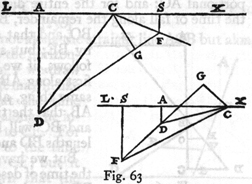THEOREM X, PROPOSITION X

The times of descent along inclined planes of the same height, but of different slope, are to each other as the lengths of these planes; and this is true whether the motion starts from rest or whether it is preceded by a fall from a constant height.

Let the paths of descent be along ABC and ABD to the horizontal plane DC so that the falls along BD and BC are preceded by the fall along AB; then, I say, that the time of descent along BD is to the time of descent along BC as the length BD is to BC.  Draw the horizontal line AF and extend DB until it cuts this  line at F; let FE be a mean proportional between DF and FB; draw EO parallel to DC; then AO will be a mean proportional between CA and AB.  If now we represent the time of fall along (198) AB by the length AB, then the time of descent along FB will be represented by the distance FB; so also the time of fall through the entire distance AC will be represented by the mean proportional AO: and for the entire distance FD by FE.  Hence the time of fall along the remainder, BC, will be represented by BO, and that along the remainder, BD, by BE; but since BE:BO=BD:BC, it follows, if we allow the bodies to fall first along AB and FB, or, what is the same thing, along the common stretch AB, that the times of descent along BD and BC will be to each other as the lengths BD and BC.But we have previously proven that the time of descent, from rest at B, along BD is to the time along BC in the ratio which the length BD bears to BC.  Hence the times of descent along different planes of constant height are to each other as the lengths of these planes, whether the motion starts from rest or is preceded by a fall from a constant height.  Q.E.D.

THEOREM XI, PROPOSITION XI

If a plane be divided into any two parts and if motion along it starts from rest, then the time of descent along the first part is to the time of descent along the remainder as the length of this first part is to the excess of a mean proportional between this first part and the entire length over this first part.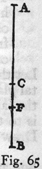Let the fall take place, from rest at A, through the entire distance AB which is divided at any point C; also let AF be a mean proportional between the entire length BA and the first part AC; then CF will denote the excess of the mean proportional FA over the first part AC.  Now, I say, the time of descent along AC will be to the time of subsequent fall through CB as the length AC is to CF.  This is evident, because the time along AC is to the time along the entire distance AB as AC is to the mean proportional AF.  (199) Therefore, dividendo, the time along AC will be to the time along the remainder CB as AC is to CF.  If we agree to represent the time along AC by the length AC then the time along CB will be represented by CF.  Q.E.D.  

In case the motion is not along the straight line ACB but along the broken line ACD to the horizontal line BD, and if from F we draw the horizontal line FE, it may in like manner be proved that the time along AC is to the time along the inclined line CD as AC is to CE.  For the time along AC is to the time along CB as AC is to CF; but it has already been shown that the time along CB, after the fall through the distance AC, is to the time along CD, after descent through the same distance AC, as CB is to CD, or, as CF is to CE; therefore, ex aequali, the time along AC will be to the time along CD as the length AC is to the length CE.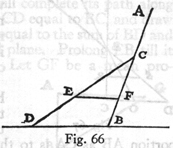THEOREM XII, PROPOSITION XII

If a vertical plane and any inclined plane are limited by two horizontals, and if we take mean proportionals between the lengths of these planes and those portions of them which lie between their point of intersection and the upper horizontal, then the time of fall along the perpendicular bears to the time required to traverse the upper part of the perpendicular plus the time required to traverse the lower part of the intersecting plane the same ratio which the entire length of the vertical bears to a length which is the sum of the mean proportional on the vertical plus the excess of the entire length of the inclined plane over its mean proportional.

Let AF and CD be two horizontal planes limiting the vertical plane AC and the inclined plane DF; let the two last-mentioned planes intersect at B.  Let AR be a mean proportional between (200) the entire vertical AC and its upper part AB; and let FS be a mean proportional between FD and its upper part FB.  Then, I say, the time of fall along the entire vertical path AC bears to the time of fall along its upper portion AB plus the time of fall along the lower part of the inclined plane, namely, BD, the same ratio which the length AC bears to the mean proportional on the vertical, namely, AR, plus the length SD which is the excess of the entire plane DF over its mean proportional FS.

Join the points R and S giving a horizontal line RS.  Now since the time of fall through the entire distance AC is to the time along the portion AB as CA is to the mean proportional AR it follows that, if we agree to represent the time of fall through AC by the distance AC, the time of fall through the distance AB will be represented by AR; and the time of descent through the remainder, BC, will be represented by RC.  But, if the time along AC is taken to be equal to the length AC, then the time along FD will be equal to the distance FD; and we may likewise infer that the time of descent along BD, when preceded by a fall along FB or AB, is numerically equal to the distance DS.  Therefore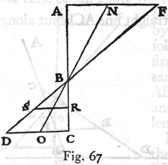the time required to fall along the path AC is equal to AR plus RC; while the time of descent along the broken line ABD will be equal to AR plus SD.  Q.E.D.

The same thing is true if, in place of a vertical plane, one takes any other plane, as for instance NO; the method of proof is also the same.

PROBLEM I, PROPOSITION XIII

Given a perpendicular line of limited length, it is required to find a plane having a vertical height equal to the given perpendicular and so inclined that a body, having fallen from rest along the perpendicular, will make its descent (201) along the inclined plane in the same time which it occupied in falling through the given perpendicular.

Let AB denote the given perpendicular: prolong this line to C making BC equal to AB, and draw the horizontal lines CE and AG.  It is required to draw a plane from B to the horizontal line CE such that after a body starting from rest at A has fallen through the distance AB, it will complete its path along this plane in an equal time.  Lay off CD equal to BC, and draw the line BD.  Construct the line BE equal to the sum of BD and DC; then, I say, BE is the required plane.  Prolong EB till it intersects the horizontal AG at G.  Let GF be a mean proportional between GE and GB; then EF:FB = EG:GF, and EF2:FB2 = EG2:GF2 = EG:GB.  But EG is twice GB; hence the square of EF is twice the square of FB; so also is the square of DB twice the square of BC.  Consequently EF:FB = DB:BC, and componendo et permutando, EB:DB + BC = BF:BC.  But EB = DB + BC; hence BF = BC = BA.  If we agree that the length AB shall represent the time of fall along the line AB, then GB will represent the time of descent along GB, and GF the time along the entire distance GE; therefore BF will represent the time of descent along the difference of these paths, namely, BE, after fall from G or from A.  Q. E. F.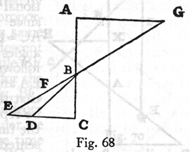PROBLEM II, PROPOSITION XIV

Given an inclined plane and a perpendicular passing through it, to find a length on the upper part of the perpendicular through which a body will fall from rest in the same time which is required to traverse the inclined plane after fall through the vertical distance just determined.

Let AC be the inclined plane and DB the perpendicular.  It is required to find on the vertical AD a length which will be (202) traversed by a body, falling from rest, in the same time which is needed by the same body to traverse the plane AC after the aforesaid fall.  Draw the horizontal CB; lay off AE such that BA + 2AC:AC = AC:AE, and lay off AR such that BA:AC = EA:AR.  From R draw RX perpendicular to DB; then, I say, X is the point sought.  For since BA + 2AC:AC = AC:AE it follows, dividendo, that BA + AC:AC = CE:AE.  And since BA:AC = EA:AR, we have, componendo, BA + AC:AC = ER:RA.  But BA + AC:AC = CE:AE, hence CE:EA = ER:RA = sum of the antecedents: sum of the consequents = CR:RE.  Thus RE is seen to be a mean proportional between CR and RA.  Moreover since it has been assumed that BA:AC = EA:AR, and since by similar triangles we have BA:AC = XA:AR, it follows that EA:AR = XA:AR.  Hence EA and XA are equal.  But if we agree that the time of fall through RA shall be represented by the length RA, then the time of fall along RC will be represented by the length RE which is a mean proportional between RA and RC; likewise AE will represent the time of descent along AC after descent along RA or along AX.  But the time of fall through XA is represented by the length XA, while RA represents the time through RA.  But it has been shown that XA and AE are equal.  Q. E. F.PROBLEM III, PROPOSITION XV

Given a vertical line and a plane inclined to it, it is required to find a length on the vertical line below its point of intersection which will be traversed in the same time as the inclined plane, each of these motions having been preceded by a fall through the given vertical line.

Let AB represent the vertical line and BC the inclined plane; it is required to find a length on the perpendicular below its point of intersection, which after a fall from A will be traversed in the (203) same time which is needed for BC after an identical fall from A.  Draw the horizontal AD, intersecting the prolongation of CB at D; let DE be a mean proportional between CD and DB; lay  off BF equal to BE; also let AG be a third proportional to BA and AF.  Then, I say, BG is the distance which a body, after falling through AB, will traverse in the same time which is needed for the plane BC after the same preliminary fall.  For if we assume that the time of fall along AB is represented by AB, then the time for DB will be represented by DB.  And since DE is a mean proportional between BD and DC, this same DE will represent the time of descent along the entire distance DC while BE will represent the time required for the difference of these paths, namely, BC, provided in each case the fall is from rest at D or at A.  In like manner we may infer that BF represents the time of descent through the distance BG after the same preliminary fall; but BF is equal to BE.  Hence the problem is solved.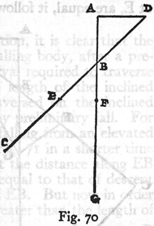THEOREM XIII, PROPOSITION XVI

If a limited inclined plane and a limited vertical line are drawn from the same point, and if the time required for a body, starting from rest, to traverse each of these is the same, then a body falling from any higher altitude will traverse the inclined plane in less time than is required for the vertical line.

Let EB be the vertical line and CE the inclined plane, both starting from the common point E, and both traversed in equal times by a body starting from rest at E; extend the vertical line upwards to any point A, from which falling bodies are allowed to start.  Then, I say that, after the fall through AE, the inclined plane EC will be traversed in less time than the perpendicular (204) EB.  Join CB, draw the horizontal AD, and prolong CE backwards until it meets the latter in D; let DF be a mean proportional between CD and DE while AG is made a mean proportional between BA and AE.  Draw FG and DG; then  since the times of descent along EC and EB, starting from rest at E, are equal, it follows, according to Corollary II of Proposition VI that the angle at C is a right angle; but the angle at A is also a right angle and the angles at the vertex E are equal; hence the triangles AED and CEB are equiangular and the sides about the equal angles are proportional; hence BE:EC = DE: EA.  Consequently the rectangle BE. EA is equal to the rectangle CE. ED; and since the rectangle CD. DE exceeds the rectangle CE. ED by the square of ED, and since the rectangle BA. AE exceeds the rectangle BE. EA by the square of EA, it follows that the excess of the rectangle CD. DE over the rectangle BA. AE, or what is the same thing, the excess of the square of FD over the square of AG, will be equal to the excess of the square of DE over the square of AE, which excess is equal to the square of AD.  Therefore FD2 = GA2 + AD2 = GD2.  Hence DF is equal to DG, and the angle DGF is equal to the angle DFG while the angle EGF is less than the angle EFG, and the opposite side EF is less than the opposite side EG.  If now we agree to represent the time of fall through AE by the length AE, then the time along DE will be represented by DE.  And since AG is a mean proportional between BA and(205) AE, it follows that AG will represent the time of fall through the total distance AB, and the difference EG will represent the time of fall, from rest at A, through the difference of path EB.

In like manner EF represents the time of descent along EC, starting from rest at D or falling from rest at A.  But it has been shown that EF is less than EG; hence follows the theorem.

COROLLARY

From this and the preceding proposition, it is clear that the vertical distance covered by a freely falling body, after a preliminary fall, and during the time-interval required to traverse an inclined plane, is greater than the length of the inclined plane, but less than the distance traversed on the inclined plane during an equal time, without any preliminary fall.  For since we have just shown that bodies falling from an elevated point A will traverse the plane EC in Fig.  71 in a shorter time than the vertical EB, it is evident that the distance along EB which will be traversed during a time equal to that of descent along EC will be less than the whole of EB.  But now in order to show that this vertical distance is greater than the length of the inclined plane EC, we reproduce Fig.  70 of the preceding theorem in which the vertical length BG is traversed in the same time as BC after a preliminary fall through AB.  That BG is greater than BC is shown as follows: since BE and FB are equal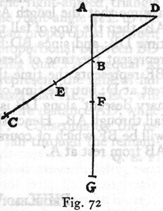while BA is less than BD, it follows that FB will bear to BA a greater ratio than EB bears to BD; and, componendo, FA will bear to BA a greater ratio than ED to DB; but FA:AB = GF:FB (since AF is a mean proportional between BA and AG) and in like manner ED:BD = CE:EB.  Hence GB bears to BF a greater ratio than CB bears to BE; therefore GB is greater than BC .

(206)

PROBLEM IV, PROPOSITION XVII

Given a vertical line and an inclined plane, it is required to lay off a distance along the given plane which will be traversed by a body, after fall along the perpendicular, in the same time-interval which is needed for this body to fall from rest through the given perpendicular.

Let AB be the vertical line and BE the inclined plane.  The problem is to determine on BE a distance such that a body, after falling through AB, will traverse it in a time equal to that required to traverse the perpendicular AB itself, starting from rest.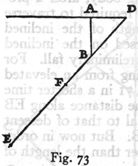Draw the horizontal AD and extend the plane until it meets this line in D.  Lay off FB equal to BA; and choose the point E such that BD:FD = DF:DE.  Then, I say, the time of descent along BE, after fall through AB, is equal to the time of fall, from rest at A, through AB.  For, if we assume that the length AB represents the time of fall through AB, then the time of fall through DB will be represented by the time DB; and since BD:FD = DF:DE, it follows that DF will represent the time of descent along the entire plane DE while BF represents the time through the portion BE starting from rest at D; but the time of descent along BE after the preliminary descent along DB is the same as that after a preliminary fall through AB.  Hence the time of descent along BE after AB will be BF which of course is equal to the time of fall through AB from rest at A.  Q. E. F.  

PROBLEM V, PROPOSITION XVIII

Given the distance through which a body will fall vertically from rest during a given time-interval, and given also a smaller time-interval, it is required to locate another [equal] (207) vertical distance which the body will traverse during this given smaller time-interval.

Let the vertical line be drawn through A, and on this line lay off the distance AB which is traversed by a body falling from rest at A, during a time which may also be represented by AB.  Draw the horizontal line CBE, and on it lay off BC to represent the given interval of time which is shorter than AB.  It is required to locate, in the perpendicular above mentioned, a distance which is equal to AB and which will be described in a time equal to BC.  Join the points A and C; then, since BC < BA, it follows that the angle BAC < angle BCA.  Construct the angle CAE equal to BCA and let E be the point where AE intersects the horizontal line; draw ED at right angles to AE, cutting the vertical at D; lay off DF equal to BA.  Then, I say, that FD is that portion of the vertical which a body starting from rest at A will traverse during the assigned time-interval BC.  For, if in the right-angled triangle AED a perpendicular be drawn from the right-angle at E to the opposite side AD, then AE will be a mean proportional between DA and AB while BE will be a mean proportional between BD and BA, or between FA and AB (seeing that FA is equal to DB); and since it has been agreed to represent the time of fall through AB by the distance AB, it follows that AE, or EC, will represent the time of fall through the entire distance AD, while EB will represent the time through AF.  Consequently the remainder BC will represent the time of fall through the remaining distance FD.  Q. E. F.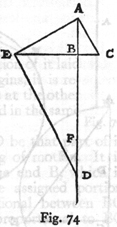PROBLEM VI, PROPOSITION XIX

Given the distance through which a body falls in a vertical line from rest and given also the time of fall, it is required to find the time in which the same body will, later, traverse (208) an equal distance chosen anywhere in the same vertical line.

On the vertical line AB, lay off AC equal to the distance fallen from rest at A, also locate at random an equal distance DB.  Let the time of fall through AC be represented by the length AC.  It is required to find the time necessary to traverse DB after fall from rest at A.  About the entire length AB describe the semicircle AEB; from C draw CE perpendicular to AB; join the points A and E; the line AE will be longer than EC; lay off EF equal to EC.  Then, I say, the difference FA will represent the time required for fall through DB.  For since AE is a mean proportional between BA and AC and since AC represents the time of fall through AC, it follows that AE will represent the time through the entire distance AB.  And since CE is a mean proportional between DA and AC (seeing that DA = BC) it follows that CE, that is, EF, will represent the time of fall through AD.  Hence the difference AF will represent the time of fall through the difference DB.  Q.E.D.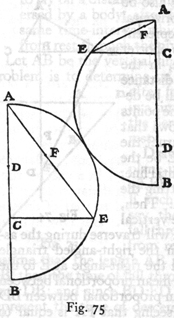COROLLARY

Hence it is inferred that if the time of fall from rest through any given distance is represented by that distance itself, then the time of fall, after the given distance has been increased by a certain amount, will be represented by the excess of the mean proportional between the increased distance and the original distance over the mean proportional between the original distance and the increment.  Thus, for instance, if we agree that (209) AB represents the time of fall, from rest at A, through the distance AB, and that AS is the increment, the time required to traverse AB, after fall through SA, will be the excess of the mean proportional between SB and BA over the mean proportional between BA and AS.PROBLEM VII, PROPOSITION XX

Given any distance whatever and a portion of it laid off from the point at which motion begins, it is required to find another portion which lies at the other end of the distance and which is traversed in the same time as the first given portion.

Let the given distance be CB and let CD be that part of it which is laid off from the beginning of motion.  It is required to find another part, at the end B, which is traversed in the same time as the assigned portion CD.  Let BA be a mean proportional between BC and CD; also let CE be a third proportional to BC and CA.  Then, I say, EB will be the distance which, after fall from C, will be traversed in the same time as CD itself.  For if we agree that CB shall represent the time through the entire distance CB, then BA (which, of course, is a mean proportional between BC and CD) will represent the time along CD; and since CA is a mean proportional between BC and CE, it follows that CA will be the time through CE; but the total length CB represents the time through the total distance CB.  Therefore the difference BA will be the time along the difference of distances, EB, after falling from C; but this same BA was the time of fall through CD.  Consequently the distances CD and EB are traversed, from rest at A, in equal times.  Q. E. F.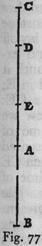THEOREM XIV, PROPOSITION XXI

If, on the path of a body falling vertically from rest, one lays off a portion which is traversed in any time you please (210) and whose upper terminus coincides with the point where the motion begins, and if this fall is followed by a motion deflected along any inclined plane, then the space traversed along the inclined plane, during a time-interval equal to that occupied in the previous vertical fall, will be greater than twice, and less than three times, the length of the vertical fall.

Let AB be a vertical line drawn downwards from the horizontal line AE, and let it represent the path of a body falling from rest at A; choose any portion AC of this path.  Through C draw any inclined plane, CG, along which the motion is continued after fall through AC.  Then, I say, that the distance  traversed along this plane CG, during the time-interval equal to that of the fall through AC, is more than twice, but less than three times, this same distance AC.  Let us lay off CF equal to AC, and extend the plane GC until it meets the horizontal in E; choose G such that CE:EF = EF:EG.  If now we assume that the time of fall along AC is represented by the length AC, then CE will represent the time of descent along CE, while CF, or CA, will represent the time of descent along CG.  It now remains to be shown that the distance CG is more than twice, and less than three times, the distance CA itself.  Since CE:EF = EF:EG, it follows that CE:EF = CF:FG; but EC < EF; therefore CF will be less than FG and GC will be more than twice FC, or AC.  Again since FE < 2EC (for EC is greater than CA, or CF), we have GF less than twice FC, and also GC less than three times CF, or CA.  Q.E.D.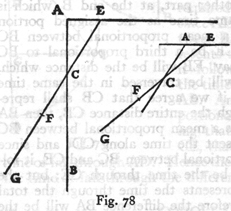This proposition may be stated in a more general form; since (211) what has been proven for the case of a vertical and inclined plane holds equally well in the case of motion along a plane of any inclination followed by motion along any plane of less steepness, as can be seen from the adjoining figure.  The method of proof is the same.



PROBLEM VIII, PROPOSITION XXII

Given two unequal time-intervals, also the distance through which a body will fall along a vertical line, from rest, during the shorter of these intervals, it is required to pass through the highest point of this vertical line a plane so inclined that the time of descent along it will be equal to the longer of the given intervals.

Let A represent the longer and B the shorter of the two unequal time-intervals, also let CD represent the length of the vertical fall, from rest, during the time B.  It is required to pass through the point C a plane of such a slope that it will be traversed in the time A.Draw from the point C to the horizontal a line CX of such a length that B:A = CD:CX.  It is clear that CX is the plane along which a body will descend in the given time A.  For it has been shown that the time of descent along an inclined plane bears to the time of fall through its vertical height the same ratio which the length of the plane bears to its vertical height.  Therefore the time along CX is to the time along CD as the length CX is to the length CD, that is, as the time-interval A is (212) to the time-interval B: but B is the time required to traverse the vertical distance, CD, starting from rest; therefore A is the time required for descent along the plane CX.

PROBLEM IX, PROPOSITION XXIII

Given the time employed by a body in falling through a certain distance along a vertical line, it is required to pass through the lower terminus of this vertical fall, a plane so inclined that this body will, after its vertical fall, traverse on this plane, during a time-interval equal to that of the vertical fall, a distance equal to any assigned distance, provided  this assigned distance is more than twice and less than three times, the vertical fall.

Let AS be any vertical line, and let AC denote both the length of the vertical fall, from rest at A, and also the time required for this fall.  Let IR be a distance more than twice and less than three times, AC.  It is required to pass a plane through the point C so inclined that a body, after fall through AC, will, during the time AC, traverse a distance equal to IR.  Lay off RN and NM each equal to AC.  Through the point C, draw a plane CE meeting the horizontal, AE, at such a point that IM:MN = AC:CE.  Extend the plane to O, and lay off CF, FG and GO equal to RN, NM, and MI respectively.  Then, I say, the time along the inclined plane CO, after fall through AC, is equal to the time of fall, from rest at A, through AC.  For since OG:GF = FC:CE, it follows, componendo, that OF:FG = OF:FC = FE:EC, and since an antecedent is to its consequent as the sum of the antecedents is to the sum of the consequents, we have OE:EF = EF:EC.  Thus EF is a mean proportional between OE and EC.  Having agreed to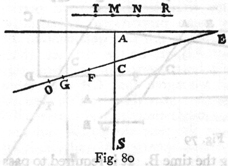(213) represent the time of fall through AC by the length AC it follows that EC will represent the time along EC, and EF the time along the entire distance EO, while the difference CF will represent the time along the difference CO; but CF = CA; therefore the problem is solved.  For the time CA is the time of fall, from rest at A, through CA while CF (which is equal to CA) is the time required to traverse CO after descent along EC or after fall through AC.  Q. E. F.

It is to be remarked also that the same solution holds if the antecedent motion takes place, not along a vertical, but along an inclined plane.  This case is illustrated in the following figure where the antecedent motion is along the inclined plane AS  underneath the horizontal AE.  The proof is identical with the preceding.

SCHOLIUM

On careful attention, it will be clear that, the nearer the given line IR approaches to three times the length AC, the nearer the inclined plane, CO, along which the second motion takes place, approaches the perpendicular along which the space traversed, during the time AC, will be three times the distance AC.  For if IR be taken nearly equal to three times AC, then IM will be almost equal to MN; and since, by construction,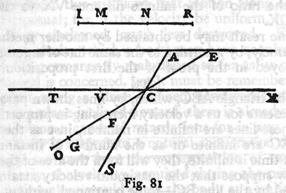(214) IM:MN = AC:CE, it follows that CE is but little greater than CA: consequently the point E will lie near the point A, and the lines CO and CS, forming a very acute angle, will almost coincide.  But, on the other hand, if the given line, IR, be only the least bit longer than twice AC, the line IM will be very short; from which it follows that AC will be very small in comparison with CE which is now so long that it almost coincides with the horizontal line drawn through C.  Hence we can infer that, if, after descent along the inclined plane AC of the adjoining figure, the motion is continued along a horizontal line, such as CT, the distance traversed by a body, during a time equal to the time of fall through AC, will be exactly twice the distance AC.  The argument here employed is the same as the preceding.  For it is clear, since OE:EF = EF:EC, that FC measures the time of descent along CO.  But, if the horizontal line TC which is twice as long as CA, be divided into two equal parts at V then this line must be extended indefinitely in the direction of X before it will intersect the line AE produced; and accordingly the ratio of the infinite length TX to the infinite length VX is the same as the ratio of the infinite distance VX to the infinite distance CX.

The same result may be obtained by another method of approach, namely, by returning to the same line of argument which was employed in the proof of the first proposition.  Let us  consider the triangle ABC, which, by lines drawn parallel to its base, represents for us a velocity increasing in proportion to the time; if these lines are infinite in number, just as the points in the line AC are infinite or as the number of instants in any interval of time is infinite, they will form the area of the triangle.  Let us now suppose that the maximum velocity attained -- that represented by the line BC -- to be continued, without acceleration and at constant value through another interval of time equal to the first.  From these velocities will be built up, in a similar manner, the area of the parallelogram ADBC, which is twice that of the triangle ABC; accordingly the distance traversed with these velocities during any given interval of time will be (215) twice that traversed with the velocities represented by the triangle during an equal interval of time.  But along a horizontal plane the motion is uniform since here it experiences neither acceleration nor retardation; therefore we conclude that the distance CD traversed during a time-interval equal to AC is twice the distance AC; for the latter is covered by a motion, starting from rest and increasing in speed in proportion to the parallel lines in the triangle, while the former is traversed by a motion represented by the parallel lines of the parallelogram which, being also infinite in number, yield an area twice that of the triangle.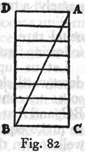Furthermore we may remark that any velocity once imparted to a moving body will be rigidly maintained as long as the external causes of acceleration or retardation are removed, a condition which is found only on horizontal planes; for in the case of planes which slope downwards there is already present a cause of acceleration, while on planes sloping upward there is retardation; from this it follows that motion along a horizontal plane is perpetual; for, if the velocity be uniform, it cannot be diminished or slackened, much less destroyed.  Further, although any velocity which a body may have acquired through natural fall is permanently maintained so far as its own nature [suapte natural] is concerned, yet it must be remembered that if, after descent along a plane inclined downwards, the body is deflected to a plane inclined upward, there is already existing in this latter plane a cause of retardation; for in any such plane this same body is subject to a natural acceleration downwards.  Accordingly we have here the superposition of two different states, namely, the velocity acquired during the preceding fall which if acting alone would carry the body at a uniform rate to infinity, and the velocity which results from a natural acceleration downwards common to all bodies.  It seems altogether reasonable, therefore, if we wish to trace the future history of a body which has descended along some inclined plane and has been deflected along some plane inclined upwards, for us to (216) assume that the maximum speed acquired during descent is permanently maintained during the ascent.  In the ascent, however, there supervenes a natural inclination downwards, namely, a motion which, starting from rest, is accelerated at the  usual rate.  If perhaps this discussion is a little obscure, the following figure will help to make it clearer.

Let us suppose that the descent has been made along the downward sloping plane AB, from which the body is deflected so as to continue its motion along the upward sloping plane BC; and first let these planes be of equal length and placed so as to make equal angles with the horizontal line GH.  Now it is well known that a body, starting from rest at A, and descending along AB, acquires a speed which is proportional to the time, which is a maximum at B, and which is maintained by the body so long as all causes of fresh acceleration or retardation are removed; the acceleration to which I refer is that to which the body would be subject if its motion were continued along the plane AB extended, while the retardation is that which the body would encounter if its motion were deflected along the plane BC inclined upwards; but, upon the horizontal plane GH, the body would maintain a uniform velocity equal to that which it had acquired at B after fall from A; moreover this velocity is such that, during an interval of time equal to the time of descent through AB, the body will traverse a horizontal distance equal to twice AB.  Now let us imagine this same body to move with the same uniform speed along the plane BC so that here also during a time-interval equal to that of descent along AB, it will traverse along BC extended a distance twice AB; but let us suppose that, at the very instant the body begins its ascent it is subjected, by its very nature, to the same influences which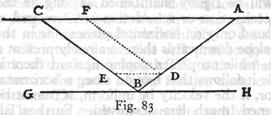(217) surrounded it during its descent from A along AB, namely, it descends from rest under the same acceleration as that which was effective in AB, and it traverses, during an equal interval of time, the same distance along this second plane as it did along AB; it is clear that, by thus superposing upon the body a uniform motion of ascent and an accelerated motion of descent, it will be carried along the plane BC as far as the point C where these two velocities become equal.

If now we assume any two points D and E, equally distant from the vertex B, we may then infer that the descent along BD takes place in the same time as the ascent along BE.  Draw DF parallel to BC; we know that, after descent along AD, the body will ascend along DF; or, if, on reaching D, the body is carried along the horizontal DE, it will reach E with the same momentum [impetus] with which it left D; hence from E the body will ascend as far as C, proving that the velocity at E is the same as that at D.

From this we may logically infer that a body which descends  along any inclined plane and continues its motion along a plane inclined upwards will, on account of the momentum acquired, ascend to an equal height above the horizontal; so that if the descent is along AB the body will be carried up the plane BC as far as the horizontal line ACD: and this is true whether the inclinations of the planes are the same or different, as in the case of the planes AB and BD.  But by a previous postulate [p.  184] the speeds acquired by fall along variously inclined planes having the same vertical height are the same.  If therefore the planes EB and BD have the same slope, the descent along EB will be able to drive the body along BD as far as D; and since this propulsion comes from the speed acquired on reaching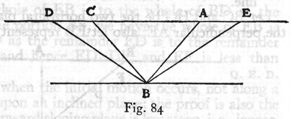(218) the point B, it follows that this speed at B is the same whether the body has made its descent along AB or EB.  Evidently then the body will be carried up BD whether the descent has been made along AB or along EB.  The time of ascent along BD is however greater than that along BC, just as the descent along EB occupies more time than that along AB; moreover it has been demonstrated that the ratio between the lengths of these times is the same as that between the lengths of the planes.  We must next discover what ratio exists between the distances traversed in equal times along planes of different slope, but of the same elevation, that is, along planes which are included between the same parallel horizontal lines.  This is done as follows:

THEOREM XV, PROPOSITION XXIV

Given two parallel horizontal planes and a vertical line connecting them; given also an inclined plane passing through the lower extremity of this vertical line; then, if a body fall freely along the vertical line and have its motion reflected along the inclined plane, the distance which it will traverse along this plane, during a time equal to that of the vertical fall, is greater than once but less than twice the vertical line.

Let BC and HG be the two horizontal planes, connected by the perpendicular AE; also let EB represent the inclined plane along which the motion takes place after the body has fallen along AE and has been reflected from E towards B.  Then, I say, that, during a time equal to that of fall along AE, the body will ascend the inclined plane through a distance which is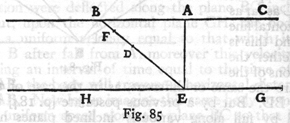(219) greater than AE but less than twice AE.  Lay off ED equal to AE and choose F so that EB:BD = BD:BF.  First we shall  show that F is the point to which the moving body will be carried after reflection from E towards B during a time equal to that of fall along AE; and next we shall show that the distance EF is greater than EA but less than twice that quantity.

Let us agree to represent the time of fall along AE by the length AE, then the time of descent along BE, or what is the same thing, ascent along EB will be represented by the distance EB.

Now, since DB is a mean proportional between EB and BF, and since BE is the time of descent for the entire distance BE, it follows that BD will be the time of descent through BF, while the remainder DE will be the time of descent along the remainder FE.  But the time of descent along the fall from rest at B is the same as the time of ascent from E to F after reflection from E with the speed acquired during fall either through AE or BE.  Therefore DE represents the time occupied by the body in passing from E to F, after fall from A to E and after reflection along EB.  But by construction ED is equal to AE.  This concludes the first part of our demonstration.

Now since the whole of EB is to the whole of BD as the portion DB is to the portion BF, we have the whole of EB is to the whole of BD as the remainder ED is to the remainder DF; but EB > BD and hence ED > DF, and EF is less than twice DE or AE.  Q. E. . D.

The same is true when the initial motion occurs, not along a perpendicular, but upon an inclined plane: the proof is also the same provided the upward sloping plane is less steep, i.e., longer, than the downward sloping plane.

THEOREM XVI, PROPOSITION XXV

If descent along any inclined plane is followed by motion along a horizontal plane, the time of descent along the inclined plane bears to the time required to traverse any assigned length of the horizontal plane the same ratio which (220) twice the length of the inclined plane bears to the given horizontal length.

Let CB be any horizontal line and AB an inclined plane; after descent along AB let the motion continue through the assigned horizontal distance BD.  Then, I say, the time of descent along AB bears to the time spent in traversing BD the same ratio which twice AB bears to BD.  For, lay off BC equal to twice AB then it follows, from a previous proposition, that the time of descent along AB is equal to the time required to traverse BC; but the time along BC is to the time along DB as the length CB is to the length BD.  Hence the time of descent along AB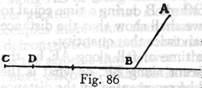is to the time along BD as twice the distance AB is to the distance BD.  Q.E.D.

PROBLEM X, PROPOSITION XXVI

Given a vertical height joining two horizontal parallel lines; given also a distance greater than once and less than twice this vertical height, it is required to pass through the foot of the given perpendicular an inclined plane such that, after fall through the given vertical height, a body whose motion is deflected along the plane will traverse the assigned distance in a time equal to the time of vertical fall.

Let AB be the vertical distance separating two parallel horizontal lines AO and BC; also let FE be greater than once and less than twice BA.  The problem is to pass a plane through B, extending to the upper horizontal line, and such that a body, after having fallen from A to B, will, if its motion be deflected along the inclined plane, traverse a distance equal to EF in a time equal to that of fall along AB.  Lay off ED equal to AB; then the remainder DF will be less than AB since the entire length EF is less than twice this quantity; also lay off DI equal to DF, and choose the point X such that EI:ID = DF:FX; from B, draw the plane BO equal in length to EX.  Then, I say, (221) that the plane BO is the one along which, after fall through AB, a body will traverse the assigned distance FE in a time equal to the time of fall through AB.  Lay off BR and RS equal to ED and DF respectively; then since EI:ID = DF:FX, we have, componendo, ED:DI = DX:XF = ED:DF = EX:XD = BO:OR = RO:OS.  If we represent the time of fall along AB by the length AB, then OB will represent the time of descent along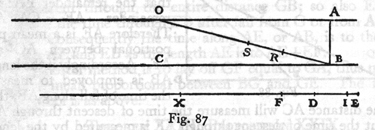OB, and RO will stand for the time along OS, while the remainder BR will represent the time required for a body starting from rest at O to traverse the remaining distance SB.  But the time of descent along SB starting from rest at O is equal to the time of ascent from B to S after fall through AB.  Hence BO is that plane, passing through B, along which a body, after fall through AB, will traverse the distance BS, equal to the assigned distance EF, in the time-interval BR or BA.  Q. E. F.

THEOREM XVII, PROPOSITION XXVII

If a body descends along two inclined planes of different lengths but of the same vertical height, the distance which it will traverse, in the lower part of the longer plane, during a time-interval equal to that of descent over the shorter plane, is equal to the length of the shorter plane plus a portion of it to which the shorter plane bears the same ratio which the longer plane bears to the excess of the longer over the shorter plane.

Let AC be the longer plane, AB, the shorter, and AD the common elevation; on the lower part of AC lay off CE equal (222) to AB.  Choose F such that CA:AE = CA:CA - AB = CE:EF.  Then, I say, that FC is that distance which will, after fall from A, be traversed during a time-interval equal to that required for descent along AB.  For since CA:AE = CE:EF, it follows that the remainder EA: the remainder AF = CA:AE.  Therefore AE is a mean proportional between AC and AF.  Accordingly if the length AB is employed to measure the time of fall along AB, then the distance AC will measure the time of descent through AC; but the time of descent through AF is measured by the length AE, and that through FC by EC.  Now EC = AB; and hence follows the proposition.PROBLEM XI, PROPOSITION XXVIII

Let AG be any horizontal line touching a circle; let AB be the diameter passing through the point of contact; and let AE and EB represent any two chords.  The problem is to determine what ratio the time of fall through AB bears to the time of descent over both AE and EB.  Extend BE till it meets the tangent at G, and draw AF so as to bisect the angle BAE.  Then, I say, the time through AB is to the sum of the times along AE and EB as the length AE is to the sum of the lengths AE and EF.  For since the angle FAB is equal to the angle FAE, while the angle EAG is equal to the angle ABF it follows that the entire angle GAF is equal to the sum of the angles FAB and ABF.  But the angle GFA is also equal to the sum of these two angles.  Hence the length GF is equal to the length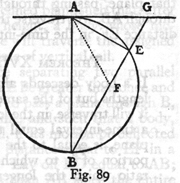(223) GA; and since the rectangle BG. GE is equal to the square of GA, it will also be equal to the square of GF, or BG:GF = GF:GE.  If now we agree to represent the time of descent along AE by the length AE, then the length GE will represent the time of descent along GE, while GF will stand for the time of descent through the entire distance GB; so also EF will denote the time through EB after fall from G or from A along AE.  Consequently the time along AE, or AB, is to the time along AE and EB as the length AE is to AE + EF.  Q.E.D.

A shorter method is to lay off GF equal to GA, thus making GF a mean proportional between BG and GE.  The rest of the proof is as above.

THEOREM XVIII, PROPOSITION XXIX

Given a limited horizontal line, at one end of which is erected a limited vertical line whose length is equal to one-half the given horizontal line; then a body, falling through this given height and having its motion deflected into a horizontal direction, will traverse the given horizontal distance and vertical line in less time than it will any other vertical distance plus the given horizontal distance.Let BC be the given distance in a horizontal plane; at the end B erect a perpendicular, on which lay off BA equal to half BC.  Then, I say, that the time required for a body, starting from rest at A, to traverse the two distances, AB and BC, is the least of all possible times in which this same distance BC together with a vertical portion, whether greater or less than AB, can be traversed.

Lay off EB greater than AB, as in the first figure, and less (224) than AB, as in the second.  It must be shown that the time required to traverse the distance EB plus BC is greater than that required for AB plus BC.  Let us agree that the length AB shall represent the time along AB, then the time occupied in traversing the horizontal portion BC will also be AB, seeing that BC = 2AB; consequently the time required for both AB and BC will be twice AB.  Choose the point O such that EB:BO = BO:BA, then BO will represent the time of fall through EB.  Again lay off the horizontal distance BD equal to twice BE; whence it is clear that BO represents the time along BD after fall through EB.  Select a point N such that DB:BC = EB:BA = OB:BN.  Now since the horizontal motion is uniform and since OB is the time occupied in traversing BD, after fall from E, it follows that NB will be the time along BC after fall through the same height EB.  Hence it is clear that OB plus BN represents the time of traversing EB plus BC; and, since twice BA is the time along AB plus BC.  it remains to be shown that OB + BN > 2BA.

But since EB:BO = BO:BA, it follows that EB:BA = OB2:BA2.  Moreover since EB:BA = OB:BN it follows that OB:BN= OB2:BA2.  But OB:BN = (OB:BA)(BA:BN), and therefore AB:BN = OB:BA, that is, BA is a mean proportional between BO and BN.  Consequently OB + BN > 2BA.  Q.E.D.  

THEOREM XIX, PROPOSITION XXX

A perpendicular is let fall from any point in a horizontal line; it is required to pass through any other point in this same horizontal line a plane which shall cut the perpendicular and along which a body will descend to the perpendicular in the shortest possible time.  Such a plane will cut from the perpendicular a portion equal to the distance of the assumed point in the horizontal from the upper end of the perpendicular.

Let AC be any horizontal line and B any point in it from which is dropped the vertical line BD.  Choose any point C in the horizontal line and lay off, on the vertical, the distance BE (225) equal to BC; join C and E.  Then, I say, that of all inclined planes that can be passed through C, cutting the perpendicular, CE is that one along which the descent to the perpendicular is accomplished in the shortest time.  For, draw the plane CF cutting the vertical above E, and the plane CG cutting the vertical below E; and draw IK, a parallel vertical line, touching at C a circle described with BC as radius.  Let EK be drawn parallel to CF, and extended to meet the tangent, after cutting the circle at L.  Now it is clear that the time of fall along LE is equal to the time along CE; but the time along KE is greater than along LE; therefore the time along KE is greater than along CE.  But the time along KE is equal to the time along CF, since they have the same length and the same slope; and, in like manner, it follows that the planes CG and IE, having the same length and the same slope, will be traversed in equal times.  Also, since HE < IE, the time along HE will be less than the time along IE.  Therefore also the time along CE (equal to the time along HE), will be shorter than the time along IE.  Q.E.D.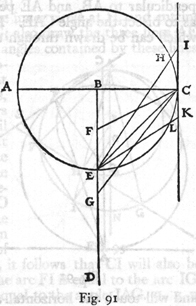THEOREM XX, PROPOSITION XXXI

If a straight line is inclined at any angle to the horizontal and if, from any assigned point in the horizontal, a plane of quickest descent is to be drawn to the inclined line, that plane will be the one which bisects the angle contained  between two lines drawn from the given point, one perpendicular (226) to the horizontal line, the other perpendicular to the inclined line.

Let CD be a line inclined at any angle to the horizontal AB; and from any assigned point A in the horizontal draw AC perpendicular to AB, and AE perpendicular to CD; draw FA so as to bisect the angle CAE.  Then, I say, that of all the planes which can be drawn through the point A, cutting the line CD at any points whatsoever AF is the one of quickest descent [in quo tempore omnium brevissimo fiat descensus].  Draw FG parallel to AE; the alternate angles GFA and FAE will be equal; also the angle EAF is equal to the angle FAG.  Therefore the sides GF and GA of the triangle FGA are equal.  Accordingly if we describe a circle about G as center, with GA as radius, this circle will pass through the point F, and will touch the horizontal at the point A and the inclined line at F; for GFC is a right angle, since GF and AE are parallel.  It is clear therefore that all lines drawn from A to the inclined line, with the single exception of FA, will extend beyond the circumference of the circle, thus requiring more time to traverse any of them than is needed for FA.  Q.E.D.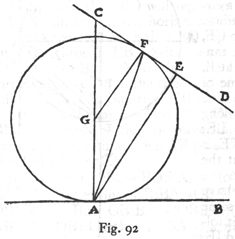LEMMA

If two circles one lying within the other are in contact, and if any straight line be drawn tangent to the inner circle, cutting the outer circle, and if three lines be drawn from the point at which the circles are in contact to three points on the tangential straight line, namely, the point of tangency on the inner circle and the two points where the (227) straight line extended cuts the outer circle, then these three lines will contain equal angles at the point of contact.

Let the two circles touch each other at the point A, the center of the smaller being at B, the center of the larger at C.  Draw  the straight line FG touching the inner circle at H, and cutting the outer at the points F and G; also draw the three lines AF, AH, and AG.  Then, I say, the angles contained by these lines, FAH and GAH, are equal.  Prolong AH to the circumference at I; from the centers of the circles, draw BH and CI; join the centers B and C and extend the line until it reaches the point of contact at A and cuts the circles at the points O and N.  But now the lines BH and CI are parallel, because the angles ICN and HBO are equal, each being twice the angle IAN.  And since BH, drawn from the center to the point of contact is perpendicular to FG, it follows that CI will also be perpendicular to FG and that the arc FI is equal to the arc IG; consequently the angle FAI is equal to the angle IAG.  Q.E.D.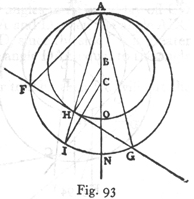THEOREM XXI, PROPOSITION XXXII

If in a horizontal line any two points are chosen and if through one of these points a line be drawn inclined towards the other, and if from this other point a straight line is drawn to the inclined line in such a direction that it cuts off from the inclined line a portion equal to the distance between the two chosen points on the horizontal line, then the time of descent along the line so drawn is less than along any other straight line drawn from the same point to the same inclined line.  Along other lines which make equal angles on opposite sides of this line, the times of descent are the same.  (228)

Let A and B be any two points on a horizontal line: through B draw an inclined straight line BC, and from B lay off a distance BD equal to BA; join the points A and D.  Then, I say, the time of descent along AD is less than along any other line drawn from A to the inclined line BC.  From the point A draw AE perpendicular to BA; and from the point D draw DE perpendicular to BD, intersecting AE at E.  Since in the isosceles triangle ABD, we have the angles BAD and BDA equal,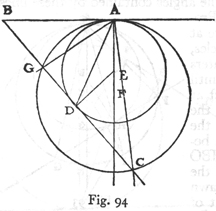their complements DAE and EDA are equal.  Hence if, with E as center and EA as radius, we describe a circle it D will pass through D and will touch the lines BA and BD at the points A and D.  Now since A is the end of the vertical line AE, the descent along AD will occupy less time than along any other line drawn from the extremity A to the line BC and extending beyond the circumference of the circle; which concludes the first part of the proposition.

If however, we prolong the perpendicular line AE, and choose any point F upon it, about which as center, we describe a circle of radius FA, this circle, AGC, will cut the tangent line in the points G and C.  Draw the lines AG and AC which will according to the preceding lemma, deviate by equal angles from the median line AD.  The time of descent along either of these lines is the same, since they start from the highest point A, and terminate on the circumference of the circle AGC.

PROBLEM XII, PROPOSITION XXXIII

Given a limited vertical line and an inclined plane of equal height, having a common upper terminal; it is required to find a point on the vertical line, extended upwards, from (229) which a body will fall and, when deflected along the inclined plane, will traverse it in the same time-interval which is required for fall, from rest, through the given vertical height.

Let AB be the given limited vertical line and AC an inclined plane having the same altitude.  It is required to find on the vertical BA, extended above A, a point from which a falling body will traverse the distance AC in the same time which is spent in falling, from rest at A, through the given vertical line AB.  Draw the line DCE at right angles to AC, and lay off CD equal to AB; also join the points A and D; then the angle ADC will be greater than the angle CAD, since the side CA is greater than either AB or CD.  Make the angle DAE equal to the angle  ADE, and draw EF perpendicular to AE; then EF will cut the inclined plane, extended both ways, at F.  Lay off AI and AG each equal to CF; through G draw the horizontal line GH.  Then, I say, H is the point sought.

For, if we agree to let the length AB represent the time of fall along the vertical AB, then AC will likewise represent the time of descent from rest at A, along AC; and since, in the right-angled triangle AEF, the line EC has been drawn from the right angle at E perpendicular to the base AF, it follows that AE will be a mean proportional between FA and AC, while CE will be a mean proportional between AC and CF, that is between CA and AI.  Now, since AC represents the time of descent from A along AC, it follows that AE will be the time along the entire distance AF, and EC the time along AI.  But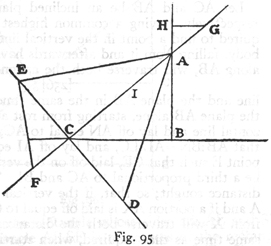(230) since in the isosceles triangle AED the side EA is equal to the side ED it follows that ED will represent the time of fall along AF, while EC is the time of fall along AI.  Therefore CD, that is AB, will represent the time of fall, from rest at A, along IF; which is the same as saying that AB is the time of fall, from G or from H, along AC.  E. F.

PROBLEM XIII, PROPOSITION XXXIV

Given a limited inclined plane and a vertical line having their highest point in common, it is required to find a point in the vertical line extended such that a body will fall from it and then traverse the inclined plane in the same time which is required to traverse the inclined plane alone starting from rest at the top of said plane.

Let AC and AB be an inclined plane and a vertical line respectively, having a common highest point at A.  It is required to find a point in the vertical line, above A, such that a body, falling from it and afterwards having its motion directed along AB, will traverse both the assigned part of the vertical  line and the plane AB in the same time which is required for the plane AB alone, starting from rest at A.  Draw BC a horizontal line and lay off AN equal to AC; choose the point L so that AB:BN = AL:LC, and lay off AI equal to AL; choose the point E such that CE, laid off on the vertical AC produced, will be a third proportional to AC and BI.  Then, I say, CE is the distance sought; so that, if the vertical line is extended above A and if a portion AX is laid off equal to CE, then a body falling from X will traverse both the distances, XA and AB, in the same time as that required, when starting from A, to traverse AB alone.

Draw XR parallel to BC and intersecting BA produced in R; next draw ED parallel to BC and meeting BA produced in D; on AD as diameter describe a semicircle; from B draw BF perpendicular to AD, and prolong it till it meets the circumference of the circle; evidently FB is a mean proportional between AB and BD, while FA is a mean proportional between (231) DA and AB.  Take BS equal to BI and FH equal to FB.  Now since AB:BD = AC:CE and since BF is a mean proportional  between AB and BD, while BI is a mean proportional between AC and CE, it follows that BA:AC = FB:BS, and since BA:AC = BA:BN = FB:BS we shall have, convertendo, BF:FS = AB:BN = AL:LC.  Consequently the rectangle formed by FB and CL is equal to the rectangle whose sides are AL and SF; moreover, this rectangle AL. SF is the excess of the rectangle AL. FB, or AI. BF, over the rectangle AI. BS, or AI. IB.  But the rectangle FB. LC is the excess of the rectangle AC. BF over the rectangle AL. BF; and moreover the rectangle AC. BF is equal to the rectangle AB. BI since BA:AC = FB:BI; hence the excess of the rectangle AB. BI over the rectangle AI. BF, or AI. FH, is equal to the excess of the rectangle AI. FH over the rectangle AI. IB; therefore twice the rectangle AI. FH is equal to the sum(232) of the rectangles AB. BI and AI. IB, or 2AI. FH = 2AI. IB + BI2.  Add AI2 to each side, then 2AI. IB+ BI2 + AI2 = AB2 = 2AI. FH+AI2.  Again add BF2 to each side, then AB2 + BF2 = AF2 = 2AI. FH+ AI2 + BF2 = 2AI. FH + AI2 + FH2.  But AF2 = 2AH. HF + AH2 + HF2; and hence 2AI. FH + AI2 + FH2 = 2AH. HF + AH2 + HF2.  Subtracting HF2 from each side we have 2AI. FH + AI2 = 2AH. HF + AH2.  Since now FH is a factor common to both rectangles, it follows that AH is equal to AI; for if AH were either greater or smaller than AI, then the two rectangles AH. HF plus the square of HA would be either larger or smaller than the two rectangles AI. FH plus the square of IA, a result which is contrary to what we have just demonstrated.

If now we agree to represent the time of descent along AB by the length AB, then the time through AC will likewise be measured by AC; and IB, which is a mean proportional between AC and CE, will represent the time through CE, or XA, from rest at X.  Now, since AF is a mean proportional between DA and AB, or between RB and AB, and since BF, which is equal to FH, is a mean proportional between AB and BD, that is between AB and AR, it follows, from a preceding proposition [Proposition XIX, corollary], that the difference AH represents the time of descent along AB either from rest at R or after fall from X, while the time of descent along AB, from rest at A, is measured by the length AB.  But as has just been shown, the time of fall through XA is measured by IB, while the time of descent along AB, after fall, through RA or through XA, is IA.  Therefore the time of descent through XA plus AB is measured by the length AB, which, of course, also measures the time of descent, from rest at A, along AB alone.  Q. E. F.  

PROBLEM XIV, PROPOSITION XXXV

Given an inclined plane and a limited vertical line, it is required to find a distance on the inclined plane which a body, starting from rest, will traverse in the same time as that needed to traverse both the vertical and the inclined plane.  (233)

Let AB be the vertical line and BC the inclined plane.  It is required to lay off on BC a distance which a body, starting from rest, will traverse in a time equal to that which is occupied by fall through the vertical AB and by descent of the plane.  Draw the horizontal line AD, which intersects at E the prolongation of the inclined plane CB; lay off BF equal to BA, and about E as center, with EF as radius describe the circle FIG.  Prolong FE until it intersects the circumference at G.  Choose a point H such that GB:BF = BH:HF.  Draw the line HI tangent to the circle at I.  At B draw the line BK perpendicular to FC, cutting the line EIL at L; also draw LM perpendicular to EL and cutting BC at M.  Then, I say, BM is the distance which a body, starting from rest at B, will traverse in the same time which is required to descend from rest at A through both distances, AB and BM.  Lay off EN equal to EL; then since GB:BF = BH:HF, we shall have, permutando, GB:BH = BF:HF, and, dividendo, GH:BH = BH:HF.  Consequently the rectangle GH. HF is equal to the square on BH; but this same rectangle is also equal to the square on HI; therefore BH is equal to HI.  Since, in the quadrilateral ILBH, the sides HB and HI are(234) equal and since the angles at B and I are right angles, it follows that the sides BL and LI are also equal: but El = EF; therefore  the total length LE, or NE, is equal to the sum of LB and EF.  If we subtract the common part EF, the remainder FN will be equal to LB: but, by construction, FB = BA and, therefore, LB = AB + BN.  If again we agree to represent the time of fall through AB by the length AB, then the time of descent along EB will be measured by EB; moreover since EN is a mean proportional between ME and EB it will represent the time of descent along the whole distance EM; therefore the difference of these distances, BM, will be traversed, after fall from EB, or AB, in a time which is represented by BN.  But having already assumed the distance AB as a measure of the time of fall through AB, the time of descent along AB and BM is measured by AB + BN.  Since EB measures the time of fall, from rest at E, along EB, the time from rest at B along BM will be the mean proportional between BE and BM, namely, BL.  The time therefore for the path AB + BM, starting from rest at A is AB + BN; but the time for BM alone, starting from rest at B, is BL; and since it has already been shown that BL = AB + BN, the proposition follows.

Another and shorter proof is the following: Let BC be the inclined plane and BA the vertical; at B draw a perpendicular to EC, extending it both ways; lay off BH equal to the excess of BE over BA; make the angle HEL equal to the angle BHE; prolong EL until it cuts BK in L; at L draw LM perpendicular to EL and extend it till it meets BC in M; then, I say, BM is the portion of BC sought.  For, since the angle MLE is a right angle, BL will be a mean proportional between MB and BE,(235) while LE is a mean proportional between ME and BE; lay off EN equal to LE; then NE = EL = LH, and HB = NE - BL.  But also HB = NE - (NB + BA); therefore BN + BA = BL.  If now we assume the length EB as a measure of the time of descent along EB, the time of descent, from rest at B, along BM will be represented by BL; but, if the descent along BM is from rest at E or at A, then the time of descent will be measured by BN; and AB will measure the time along AB.  Therefore the time required to traverse AB and BM, namely, the sum of the distances AB and BN, is equal to the time of descent, from rest at B, along BM alone.  Q. E. F.  

LEMMA

Let DC be drawn perpendicular to the diameter BA; from the extremity B draw the line BED at random; draw the line FB.  Then, I say, FB is a mean proportional between DB and BE.  Join the points E and F.  Through B, draw the tangent BG which will be parallel to CD.  Now, since the angle DBG is equal to the angle FDB, and since the alternate angle of GBD is equal to EFB, it follows that the triangles FDB and FEB are similar and hence BD:BF = FB:BE.LEMMA

Let AC be a line which is longer than DF, and let the ratio of AB to BC be greater than that of DE to EF.  Then, I say, AB is greater than DE.  For, if AB bears to BC a ratio greater than that of DE to EF, then DE will bear to some length shorter than EF, the same ratio which AB bears to BC.  Call this length EG; then since AB:BC = DE:EG, it follows, componendo et convertendo,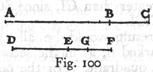(236) that CA:AB = GD:DE.  But since CA is greater than GD, it follows that BA is greater than DE.

LEMMA

Let ACIB be the quadrant of a circle; from B draw BE parallel to AC; about any point in the line BE describe a circle BOES, touching AB at B and intersecting the circumference of the quadrant at I.  Join the points C and B; draw the line CI, prolonging it to S.  Then, I say, the line CI is always less than CO.  Draw the line AI touching the circle BOE.  Then,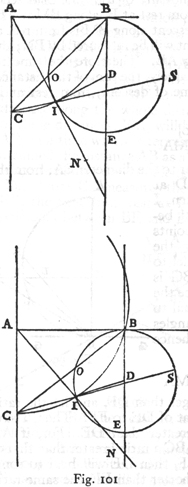if the line DI be drawn, it will be equal to DB; but, since DB touches the quadrant, DI will also be tangent to it and will be at right angles to AI; thus AI touches the circle BOE at I.  And since the angle AIC is greater than the angle ABC, subtending as it does a larger arc, it follows that the angle SIN is also greater than the angle ABC.  Wherefore the arc IES is greater than the arc BO, and the line CS, being nearer the center, is longer than CB.  Consequently CO is greater than CI, since SC:CB = OC:CI.

This result would be all the more marked if, as in the second figure, the arc BIC were less than a quadrant.  For the perpendicular DB would then cut the circle CIB; and so also would (237) DI which is equal to BD; the angle DIA would be obtuse and therefore the line AIN would cut the circle BIE.  Since the angle ABC is less than the angle AIC, which is equal to SIN, and still less than the angle which the tangent at I would make with the line SI, it follows that the arc SEI is far greater than the arc BO; whence, etc.  Q.E.D.

THEOREM XXII, PROPOSITION XXXVI

If from the lowest point of a vertical circle, a chord is drawn subtending an arc not greater than a quadrant, and if from the two ends of this chord two other chords be drawn to any point on the arc, the time of descent along the two latter chords will be shorter than along the first, and shorter also, by the same amount, than along the lower of these two latter chords.  

Let CBD be an arc, not exceeding a quadrant, taken from a vertical circle whose lowest point is C; let CD be the chord [planum elevatum] subtending this arc, and let there be two other chords drawn from C and D to any point B on the arc.  Then, I say, the time of descent along the two chords [plana] DB and BC is shorter than along DC alone, or along BC alone, starting from rest at B.  Through the point D, draw the horizontal line MDA cutting CB extended at A: draw DN and MC at right angles to MD, and BN at right angles to BD; about the right-angled triangle DBN describe the semicircle DFBN, cutting DC at F.  Choose the point O such that DO will be a mean proportional between CD and DF; in like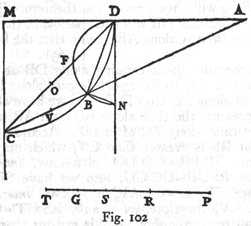(238) manner select V so that AV is a mean proportional between CA and AB.  Let the length PS represent the time of descent along the whole distance DC or BC, both of which require the same time.  Lay off PR such that CD:DO = timePS. timePR.  Then PR will represent the time in which a body, starting from D, will traverse the distance DF, while RS will measure the time in which the remaining distance, FC, will be traversed.  But since PS is also the time of descent, from rest at B, along BC, and if we choose T such that BC:CD = PS:PT then PT will measure the time of descent from A to C, for we have already shown [Lemma] that DC is a mean proportional between AC and CB.  Finally choose the point G such that CA:AV = PT:PG, then PG will be the time of descent from A to B, while GT will be the residual time of descent along BC following descent from A to B.  But, since the diameter, DN, of the circle DFN is a vertical line, the chords DF and DB will be traversed in equal times; wherefore if one can prove that a body will traverse BC, after descent along DB, in a shorter time than it will FC after descent along DF he will have proved the theorem.  But a body descending from D along DB will traverse BC in the same time as if it had come from A along AB, seeing that the body acquires the same  momentum in descending along DB as along AB.  Hence it remains only to show that descent along BC after AB is quicker than along FC after DF.  But we have already shown that GT represents the time along BC after AB; also that RS measures the time along FC after DF.  Accordingly it must be shown that RS is greater than GT, which may be done as follows: Since SP:PR = CD:DO, it follows, invertendo et convertendo, that RS:SP = OC:CD; also we have SP:PT = DC:CA.  And since TP:PG = CA:AV, it follows, invertendo, that PT:TG = AC:CV, therefore, ex aequali, RS:GT = OC:CV.  But, as we shall presently show, OC is greater than CV; hence the time RS is greater than the time GT, which was to be shown.  Now, since [Lemma] CF is greater than CB and FD smaller than BA, it follows that CD:DF > CA:AB.  But CD:DF = CO:OF, seeing that CD:DO = DO:DF; and CA:AB = CV2:VB2.  Therefore (239) CO:OF > CV:VB, and, according to the preceding lemma, CO > CV.  Besides this it is clear that the time of descent along DC is to the time along DBC as DOC is to the sum of DO and CV.

SCHOLIUM

From the preceding it is possible to infer that the path of quickest descent [lationem omnium velocissimam] from one point to another is not the shortest path, namely, a straight line, but the arc of a circle. * In the quadrant BAEC, having the side BC vertical, divide the arc AC into any number of equal parts, AD, DE, EF, FG, GC, and from C draw straight lines to the points A, D, E, F, G; draw also the straight lines AD, DE, EF, FG, GC.  Evidently descent along the path ADC is quicker  than along AC alone or along DC from rest at D.  But a body, starting from rest at A, will traverse DC more quickly than the path ADC; while, if it starts from rest at A, it will traverse the path DEC in a shorter time than DC alone.  Hence descent along the three chords, ADEC, will take less time than along the two chords DC.  Similarly, following descent along ADE, the time required to traverse EFC is less than that needed for EC alone.  Therefore descent is more rapid along the four chords ADEFC than along the three ADEC.  And finally a body, after descent along ADEF, will traverse the two chords, FGC, more quickly than FC alone.  Therefore, along the five chords, ADEFGC, descent will be more rapid than along the four, ADEFC.  Consequently(240) the nearer the inscribed polygon approaches a circle the shorter is the time required for descent from A to C.

What has been proven for the quadrant holds true also for smaller arcs; the reasoning is the same.

PROBLEM XV, PROPOSITION XXXVII

Given a limited vertical line and an inclined plane of equal altitude; it is required to find a distance on the inclined plane which is equal to the vertical line and which is traversed in an interval equal to the time of fall along the vertical line.

Let AB be the vertical line and AC the inclined plane.  We must locate, on the inclined plane, a distance equal to the vertical line AB and which will be traversed by a body starting from rest at A in the same time needed for fall along the vertical line.  Lay off AD equal to AB, and bisect the remainder DC at I.  Choose the point E such that AC:CI = CI:AE and lay off DG equal to AE.  Clearly EG is equal to AD, and also to AB.  And further, I say that EG is that distance which will be traversed by a body, starting from rest at A, in the same time which is required for that body to fall through the distance AB.  For since AC:CI = CI:AE = ID:DG, we have, convertendo, CA: AI = DI:IG.  And since the whole of CA is to the whole of AI as the portion CI is to the portion IG, it follows that the remainderIA is to the remainder AG as the whole of CA is to the whole of AI.  Thus AI is seen to be a mean proportional between CA and AG, while CI is a mean proportional between CA and AE.  If therefore the time of fall along AB is represented by the length AB, the time along AC will be represented by AC, while CI, or ID, will measure the time along AE.  Since AI is a mean proportional between CA and AG, and since CA is a (241) measure of the time along the entire distance AC, it follows that AI is the time along AG, and the difference IC is the time along the difference GC; but DI was the time along AE.  Consequently the lengths DI and IC measure the times along AE and CG respectively.  Therefore the remainder DA represents the time along EG, which of course is equal to the time along AB.  Q. E. F.

COROLLARY

From this it is clear that the distance sought is bounded at each end by portions of the inclined plane which are traversed in equal times.

PROBLEM XVI, PROPOSITION XXXVIII

Given two horizontal planes cut by a vertical line, it is required to find a point on the upper part of the vertical line from which bodies may fall to the horizontal planes and there, having their motion deflected into a horizontal direction, will, during an interval equal to the time of fall, traverse distances which bear to each other any assigned ratio of a smaller quantity to a larger.

Let CD and BE be the horizontal planes cut by the vertical ACB, and let the ratio of the smaller quantity to the larger be that of N to FG.  It is required to find in the upper part of the vertical line, AB, a point from which a body falling to the plane CD and there having its motion deflected along this plane, will traverse, during an interval equal to its time of fall a distance such that if another body, falling from this same point to the plane BE, there have its motion deflected along this plane and continued during an interval equal to its time of fall, will traverse a distance which bears to the former distance the  ratio of FG to N.  Lay off GH equal to N, and select the point L so that FH:HG = BC:CL.  Then, I say, L is the point sought.  For, if we lay off CM equal to twice CL, and draw the line LM cutting the plane BE at O, then BO will be equal to twice (242) BL.  And since FH:HG = BC:CL, we have, componendo et convertendo, HG. GF = N:GF = CL:LB = CM:BO.  It is clear that, since CM is double the distance LC, the space CM is that which a body falling from L through LC will traverse in the plane CD; and, for the same reason, since BO is twice the distance BL, it is clear that BO is the distance which a body, after fall through LB, will traverse during an interval equal to the time of its fall through LB.  Q. E. F.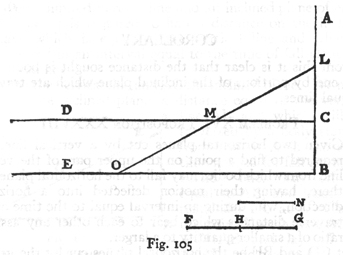SAGR.   Indeed, I think we may concede to our Academician, without flattery, his claim that in the principle [principio, i.e., accelerated motion] laid down in this treatise he has established a new science dealing with a very old subject.  Observing with what ease and clearness he deduces from a single principle the proofs of so many theorems, I wonder not a little how such a question escaped the attention of Archimedes, Apollonius, Euclid and so many other mathematicians and illustrious philosophers, especially since so many ponderous tomes have been devoted to the subject of motion.  

SALV.   There is a fragment of Euclid which treats of motion, (243) but in it there is no indication that he ever began to investigate the property of acceleration and the manner in which it varies with slope.  So that we may say the door is now opened, for the first time, to a new method fraught with numerous and wonderful results which in future years will command the attention of other minds.

SAGR.   I really believe that just as, for instance, the few properties of the circle proven by Euclid in the Third Book of his Elements lead to many others more recondite, so the principles which are set forth in this little treatise will, when taken up by speculative minds, lead to many another more remarkable result; and it is to be believed that it will be so on account of the nobility of the subject, which is superior to any other in nature.

During this long and laborious day, I have enjoyed these simple theorems more than their proofs, many of which, for their complete comprehension, would require more than an hour each; this study, if you will be good enough to leave the book in my hands, is one which I mean to take up at my leisure after we have read the remaining portion which deals with the motion of projectiles; and this if agreeable to you we shall take up tomorrow.

SALV.   I shall not fail to be with you.

END OF THE THIRD DAY.